• 表 1-1 列出了 MATLAB图像处理工具箱为描述像素值而支持的各种类。 表中的前 8 项是数值型的数据类，第 9 项称为字符类， 最后一项称为逻辑类。 uint8 和 logical 类广泛用于图像处理， 当以 TIFF 或 JPEG 图像...
图像的矩阵表示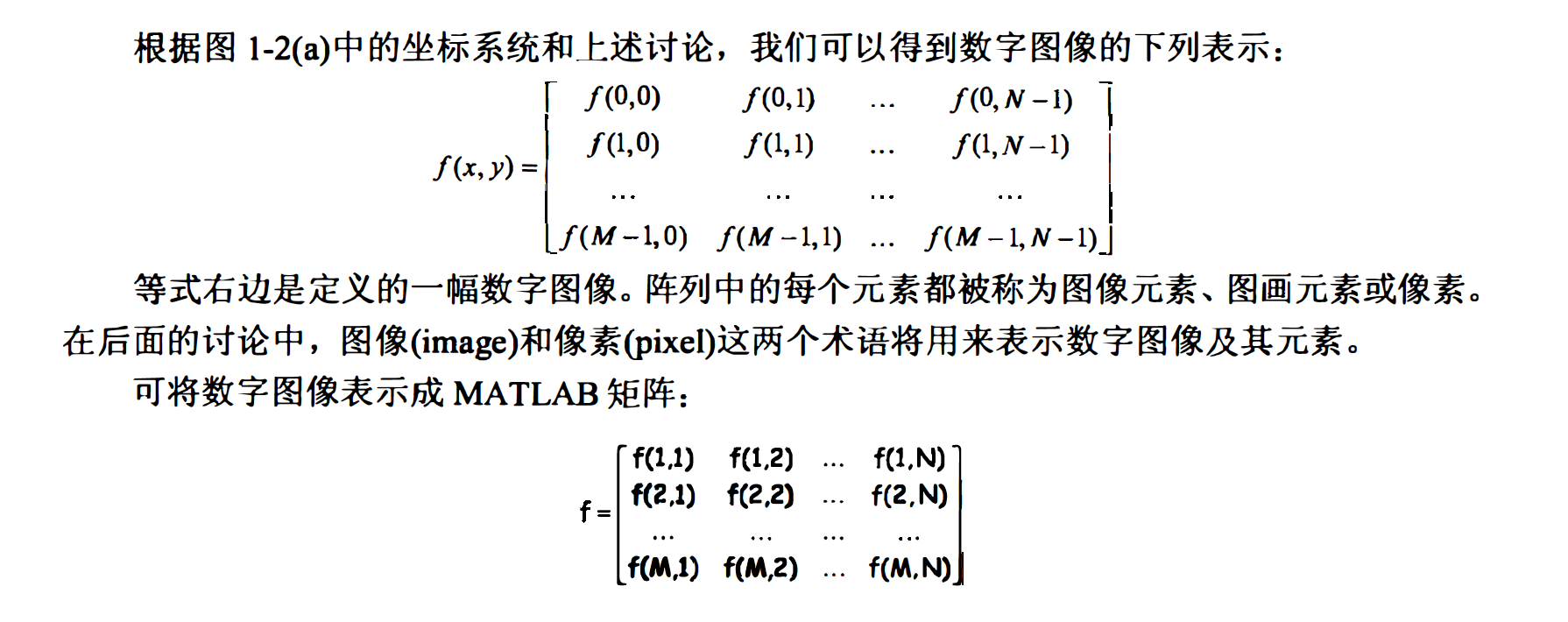类和图像类型
虽然使用的是整数坐标， 但 MATLAB 中的像素值（亮度）并未限制为整数。 表 1-1 列出了 MATLAB 和图像处理工具箱为描述像素值而支持的各种类。 表中的前 8 项是数值型的数据类，第 9 项称为字符类， 最后一项称为逻辑类。
uint8 和 logical 类广泛用于图像处理， 当以 TIFF 或 JPEG 图像文件格式读取图像时，会用到这两个类。 这两个类用1个字节表示每个像素。某些科研数据源， 比如医学成像， 要求提供超出 uint8 的动态范围：针对此类数据， 会采用 uint16 和 int16 类。 这两个类为每个矩阵元素使用2 个字节。针对计算灰度的操作， 比如傅立叶变换（见第 3 章）， 使用 double 和single 浮点类。 双精度浮点数每个数组元素使用8 个宇节， 而单精度浮点数使用 4 个字节。尽管工具箱支持 int8 、 uint32 和 int32 类， 但在图像处理中并不常用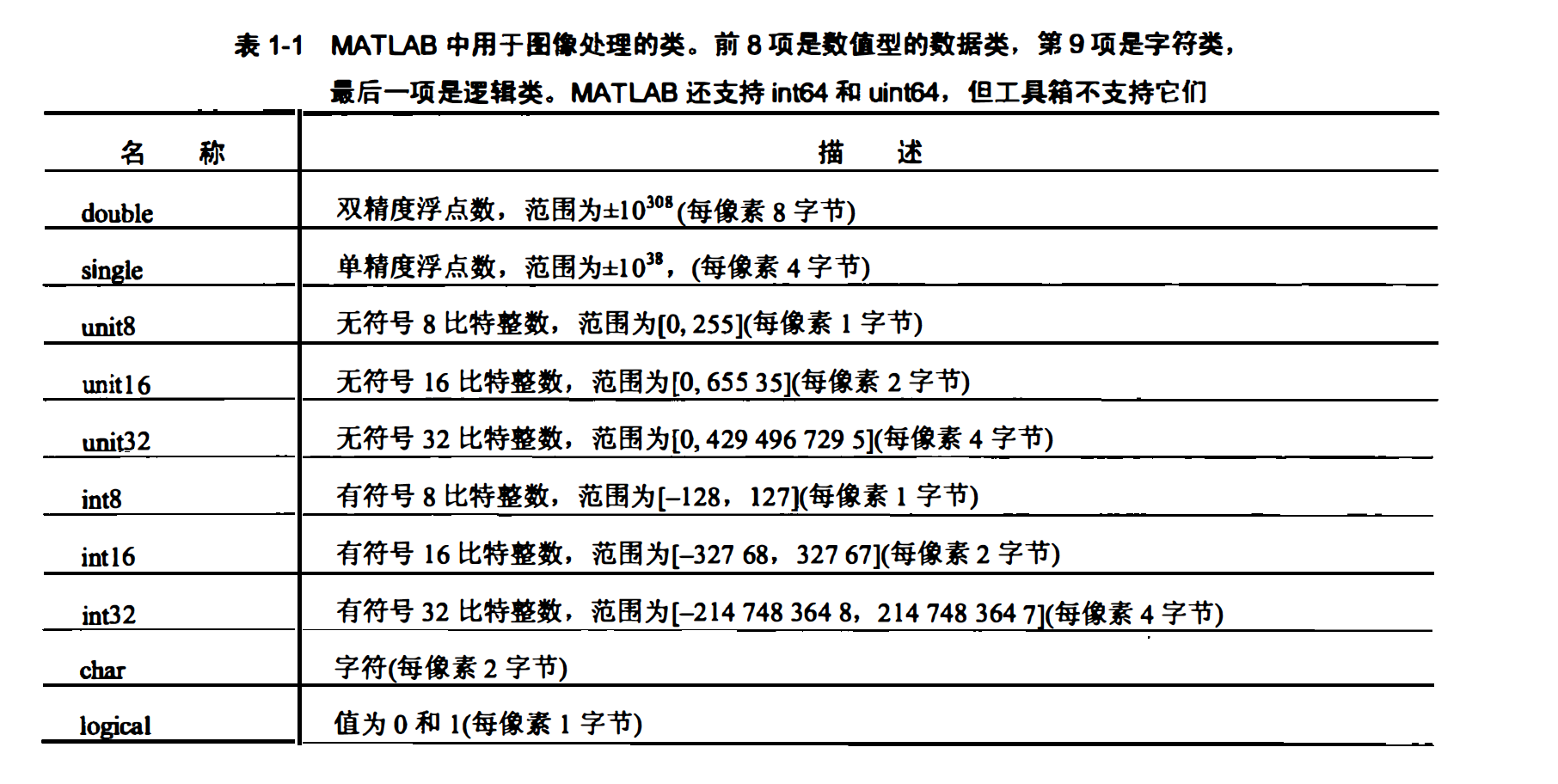算子

一般我们用字母M和N分别表示矩阵中的行与列。1x N
矩阵被称为行向量， M*1矩阵被称为列向量， 1*1矩阵则被称为标量。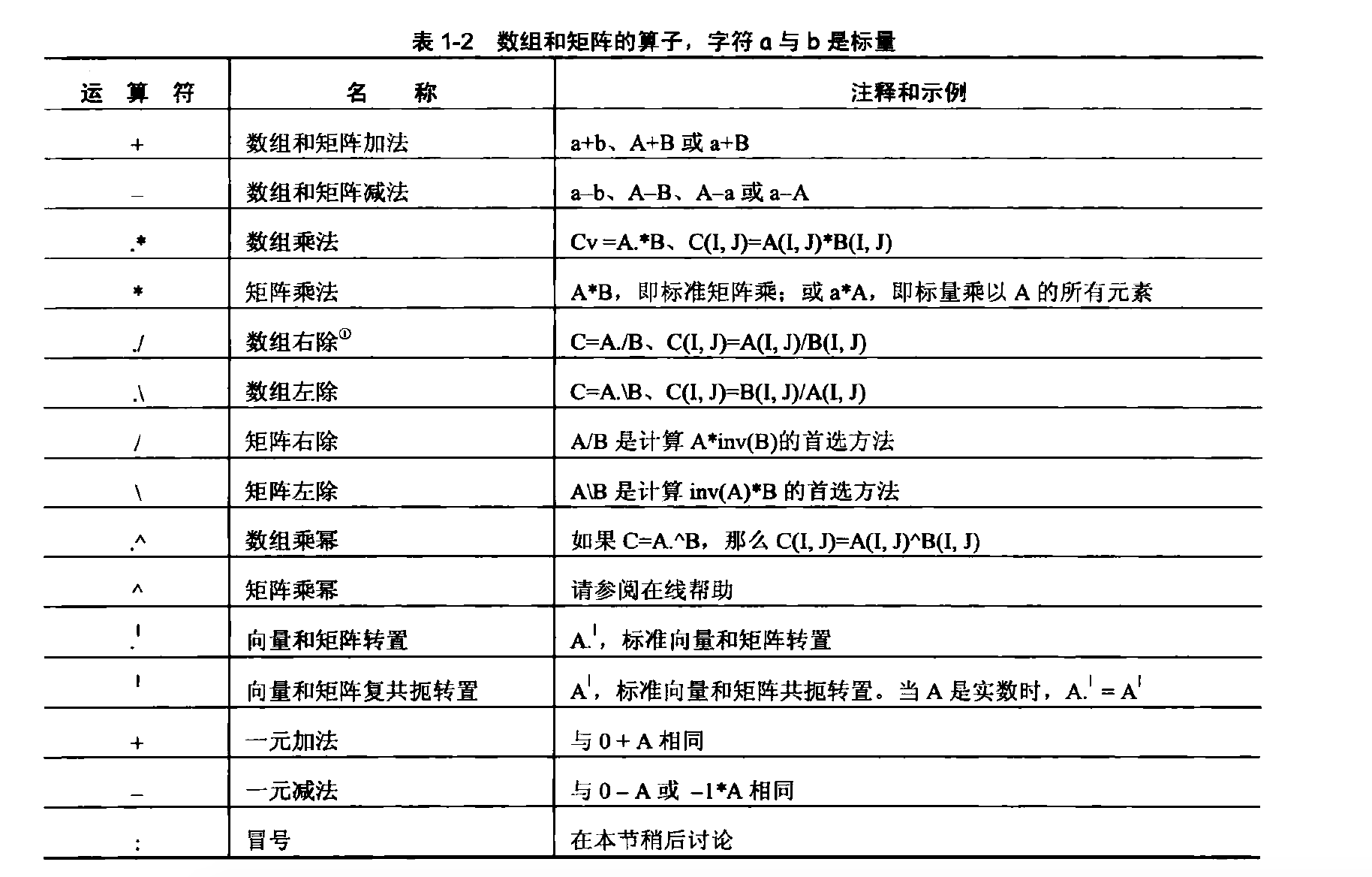Matlab 图像类型及其存储方式

在介绍数字图像的分类时， 曾提及一些主要的图像类型。 本节将介绍这些主 要的图像类型在Matlab中是如何存储和表示的， 主要包括亮度图像、 RGB 图像、 索引图像、二值图像和多帧图像。

1、亮度图像（Intensity Image)
亮度图像即灰度图像。 Matlab使用二维矩阵存储亮度图像，矩阵中的每个元素直接表示 一个像素的亮度〈灰度〉信息。 例如， 一个 200 像素× 300 像素的图像被存储为一个 200 行 300 列的矩阵，可以使用 1.1.5小节介绍的选取矩阵元素〈或子块〉的方式来选择图像中的一个像素或一个区域．

如果矩阵元素的类型是双精度的， 则元素的取值范围是从 0 到 1 ：如果是 8 位无符号整数，则取值范围从0到255。数据0表示黑色，而1（或255）表示最大亮度〈通常为白色〉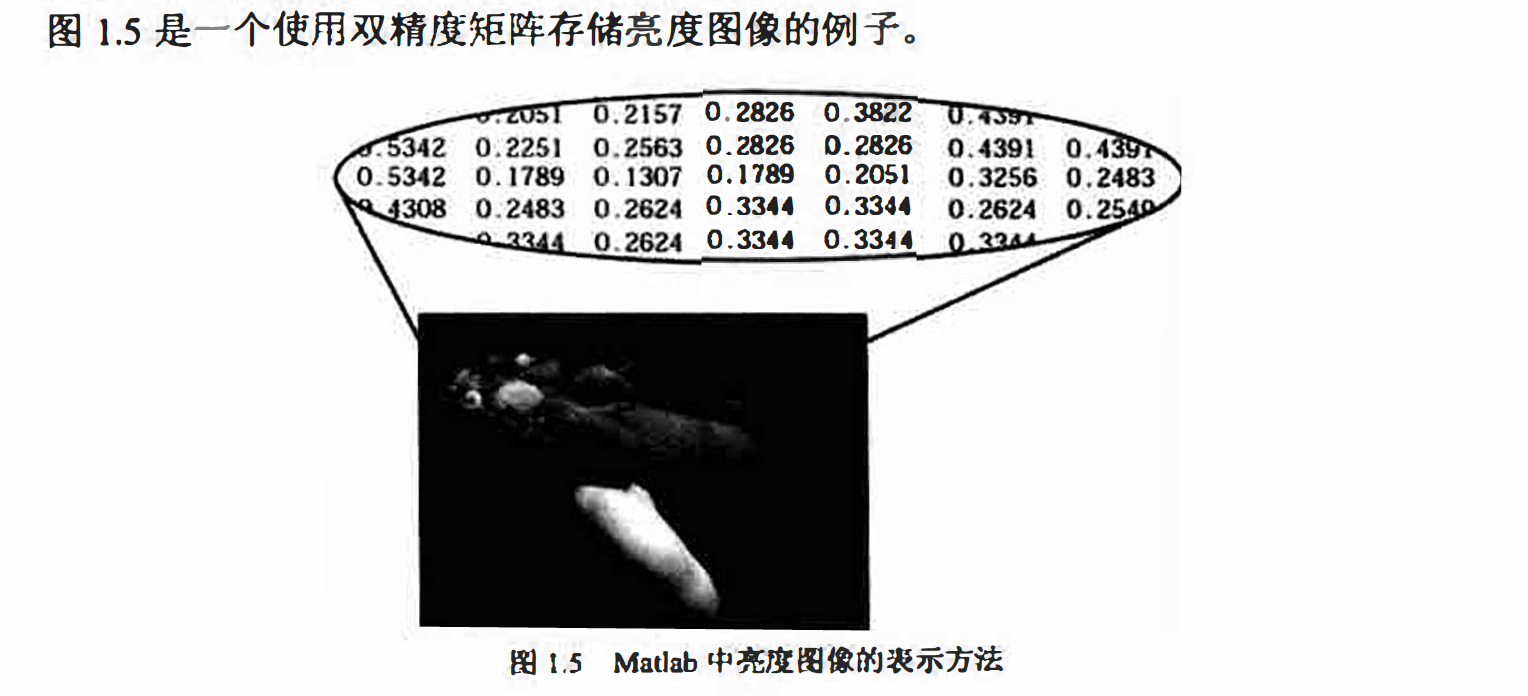2、RGB 图像（RGBlmage)
RGB 图像使用3个一组的数据表达每个像素的颜色， 即其中的红色、绿色和蓝色分量。
在Matlab 中， RGB 图像被存储在一个m ×n×3的三维数组中。对于图像中的每个像素， 存
储的三个颜色分量合成像素的最终颜色。例如， RGB 图像I 中位置在11 行40 列的像素的
RGB 值为I( 11,40.1:3 ）。或I( 11,40,:)，该像素的红色分量为I(11,40,1)，蓝色分量为I( 11,40,3 ）。
而I (:,:,1 ）则表示整个的红色分量图像．

RGB 图像同样可以由观精度数组或8 位无符号整数数组存储。图1.6 是一个使用双精度
数组存储ROB 图像的例子。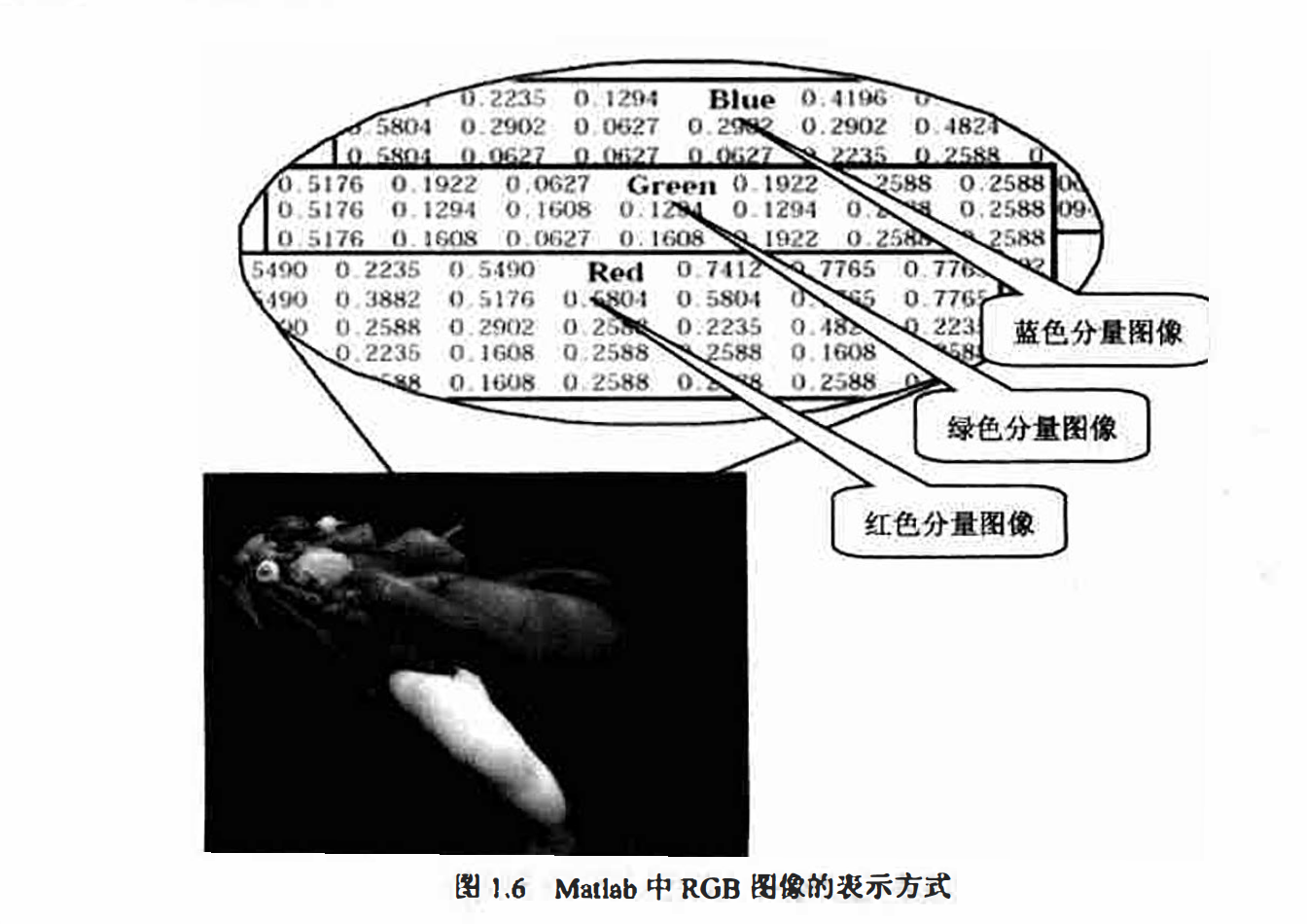3、索引图像（indexed Image)
索引图像往往包含两个数组，一个图像数据矩阵（Image Matrix ）和一个颜色索引表( Colormap ）。对应于图像中的每一个像素， 图像数据数组都包含一个指向颜色索引表的索 引值。
颜色索引表是一个m× 3 的双精度型矩阵， 每一行指定一种颜色的 3 个 RGB 分量，即color = [R G B］。 其中 R、G、 B 是实数类型的现精度数， 取值0～ 1。 0 表示全黑， 1 表示最大亮度。 图 1.7 是一个索引图像的实例， 需要注意的是， 图像中的每个像素都用整数表示， 其含义为颜色索引表中对应颜色的索引。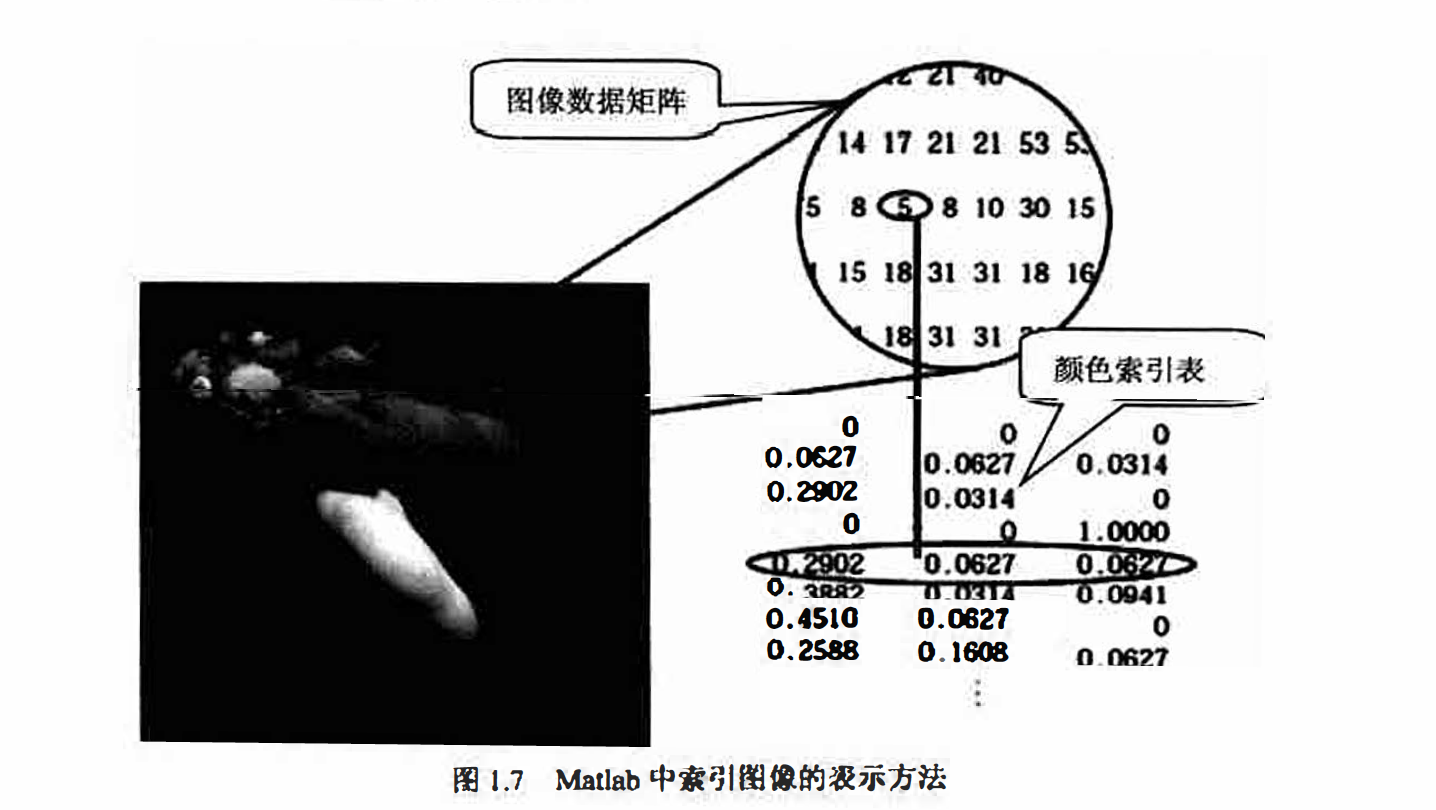图像数据矩阵和颜色索引表的关系取决于图像数据炬阵中存储的数据类型是双精度类
型还是8位无符号整数。
如果图像数据使用双精度类型存储， 则像索数据1表示颜色索引表中的第一行， 像素数
据2表示颜色索引表中的第二行， 以此类推。如果图像数据使用8位无符号整数存储， 则存
在一个额外的偏移量－1， 像素数据0表示颜色索引表中的第一行， 而1表示索引表中的第二
行， 以此类推。
8 位方式存储的图像可以支持256 种颜色（或256 级灰度〉。因1.7 中， 数据矩阵使用的
是双精度类型， 所以没有偏移量． 数据5表示颜色表中的第5种颜色。

4、二值图像（ Binary Image)
在二值图像中， 像素的颜色只有两种取值： 黑或白。Matlab 将二值图像存储为一个二维
矩阵， 每个元素的取值只有0和1两种情况，0表示黑色， 而1表示白色。
二值图像可以被看作是一种特殊的只存在黑和白两种颜色的亮度图像， 当然， 也可以将
二值图像看作是颜色索引表中只存在两种颜色〈黑和白〉的索引图像。

Matlab 中使用uint8 型的逻辑数组存储二值图像， 通过一个逻辑标志表示数据有效范围是0到1，而如果逻辑标志未被置位，则有效范围为0到255。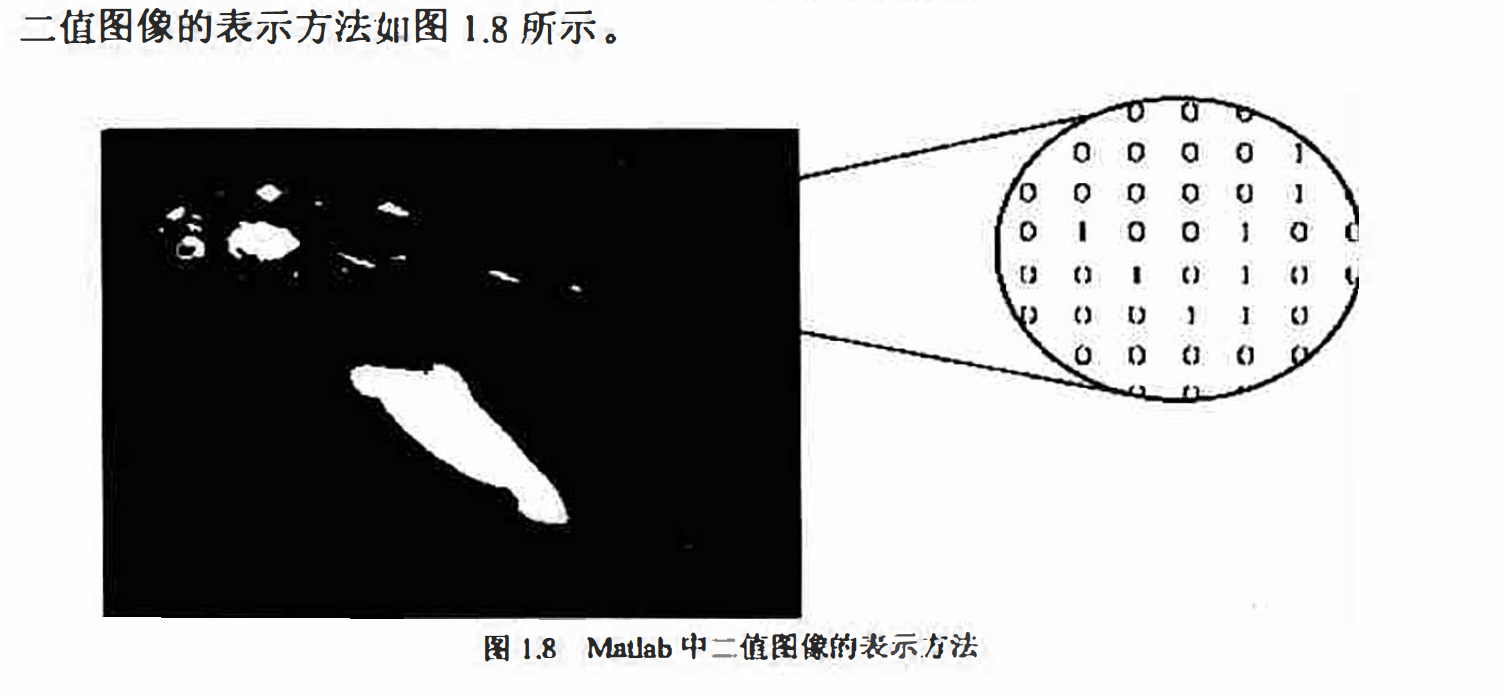5､多帧图像（ Multlframe Image Array)
实际应用中， 可能需要处理多幅按时间或视角方式连续排列的图像， 我们把这种图像称
之为多帧图像（所谓“ 帧” 就是影像动画中最小单位的单幅影像，画面〉。例如核磁共振成像数
据或视频片断. Matlab提供了在同一个矩阵中存储多帧图像的方法， 实际上就是在图像矩阵
中增加一个维度来代表时间或视角信息． 例如， 一个拥有5 张连续的400 像素× 300 像素的
RGB图像的多帧连续片断的存储方式是一个400× 300 × 3× 5 的矩阵， 一组同样大小的灰皮
图像则可以使用一个400× 300 × 1× 5 的矩阵来存储．

如果多帧图像使用索引图像的方式存储，则只有图像数据矩阵被按多帧形式存储， 而颜
色索引表只能公用。因此， 在多帧索引图像中， 所有的索引图像公用一个颜色索引表， 进而
只能使用相同的颜色组合。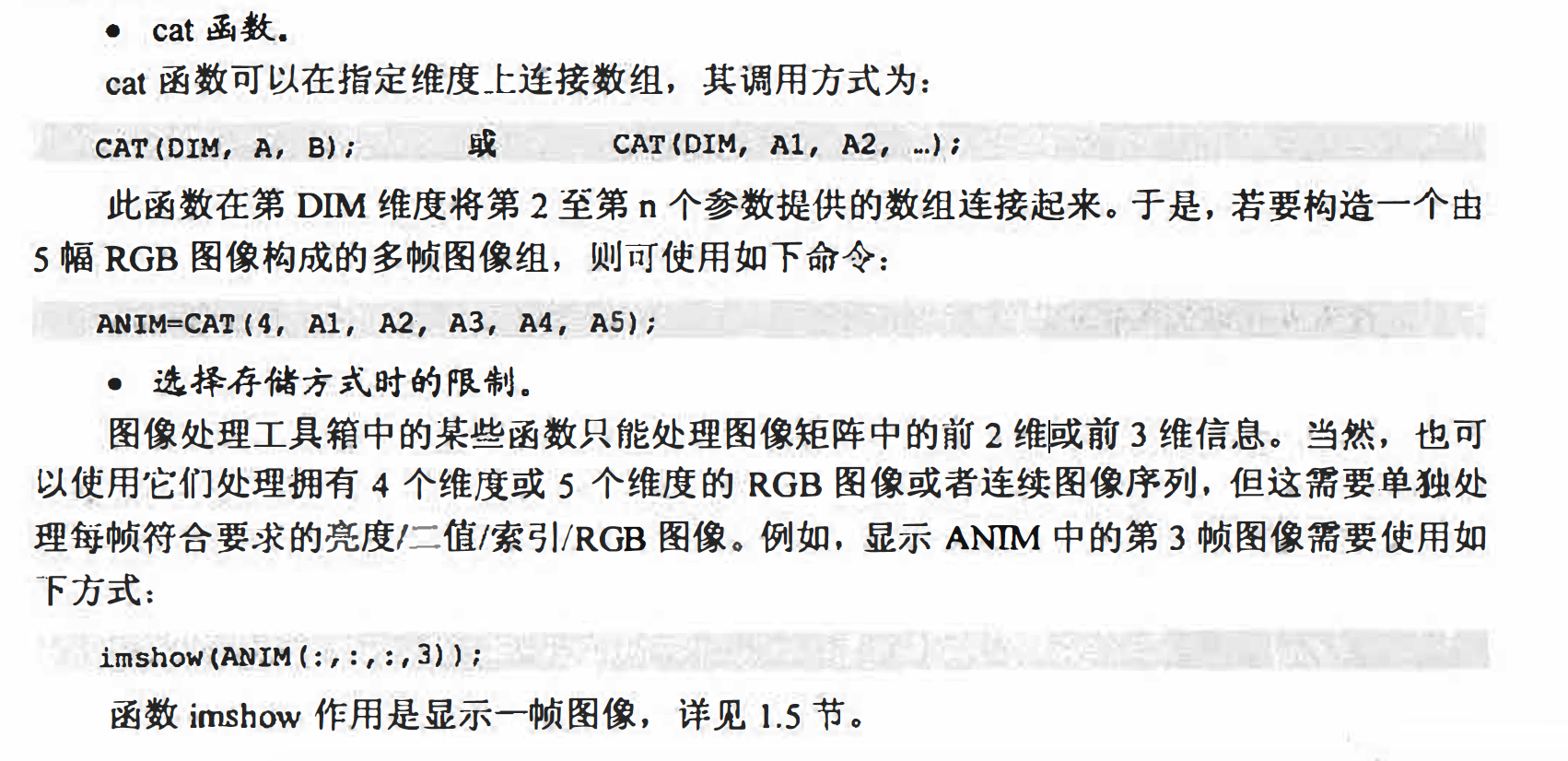默认情况下，matlab将绝大多数数据存储为双精度类型〈ω 位浮点数）以保证运算的精
确性． 而对于图像而言， 这种数据类型在图像尺寸较大时可能并不理想。例如， 一张1000
像萦见方的图像拥有一百万个像素， 如果每个像索用64位二进制数表示， 则总共需要大约
8MB的内存空间．
为了减小图像信息的空间开销， 可以将图像信息存为8 位无符号整型数（uint8）和16
位无符号整型数（ uint16 ）的数组， 这样只需要双精度浮点数八分之一或四分之一的空间。
在上述3种存储类型中双精度和 uint8 使用最多， uint16 的情况与 uint8 大致类似．

Matlab的图像转换

有时必须将图像存储格式加以转换才能使用某些图像处理函数。例如，当使用某些Matlab
内置的滤镜时， 需要将索引图像转换为RGB 图像或者灰度图像， Matlab 才会将图像滤镜应
用于图像数据本身， 而不是索引图像中的颜色索引值表〈这将产生无意义的结果〉．
Matlab 提供了一系列存储格式转换函数，如表1.11 所示．它们的名字都便于记忆，例如，
ind2gray 可以将索引图像转化为灰度图像。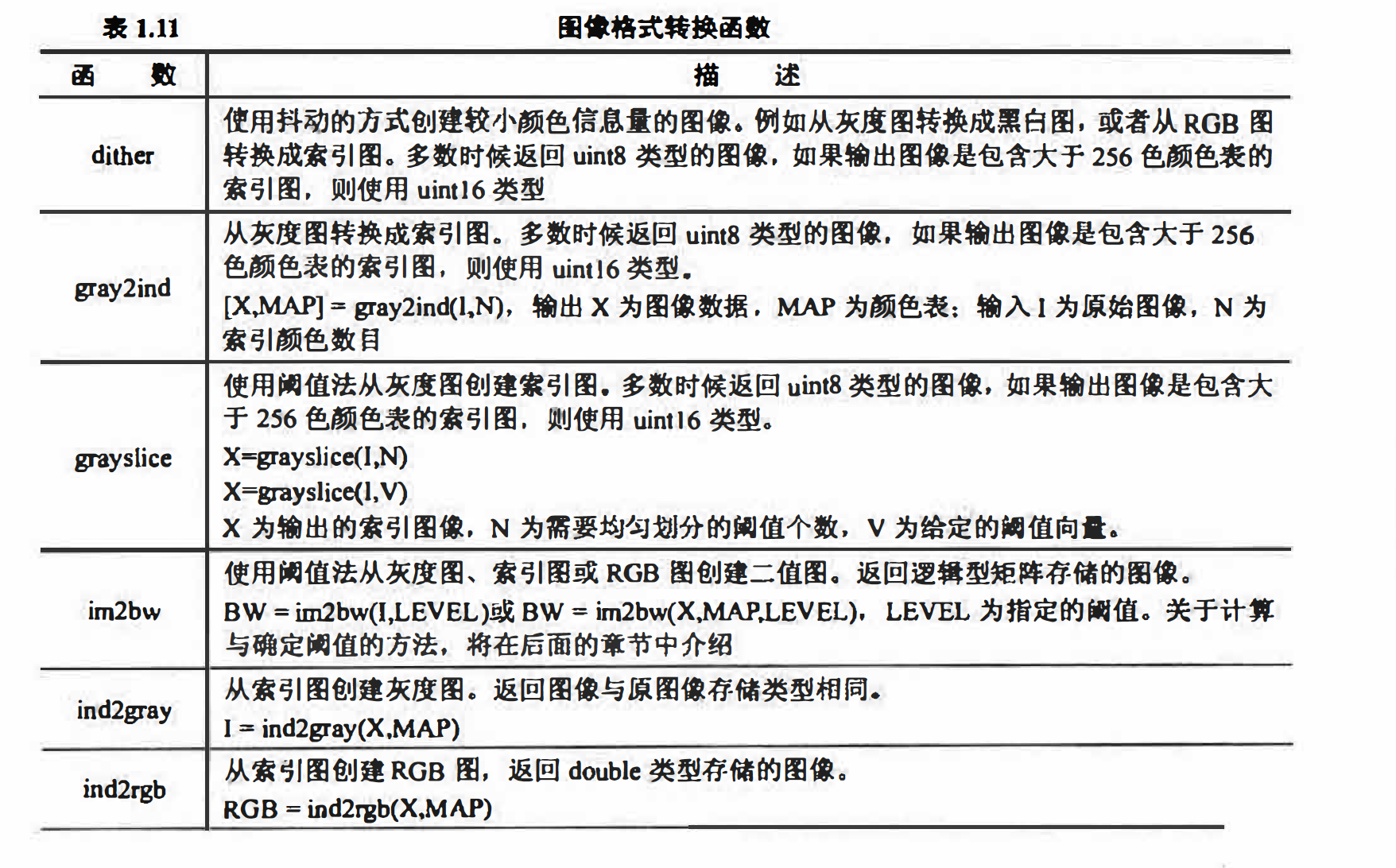也可以使用一些矩阵操作函数实现某些格式转换。例如．下面的语句可以将一幅灰度图
像转换为RGB图像．
RGBIMAGE = CAT(3, GRAY, GRAY, GRAY);

2. 图像数据类型转换
图像文件一般都为uint8类型。然而， 很多数学函数如sin等并不支持double以外的类
型， 例如， 当试图对uint8 类型直接使用sin函数进行操作时， Matlb会提示如下的错
误信息

sin(D):
H? Undehned functioii <!>i; method ’sin’ for; input a,rguments of type- ’uint8'

针对这种情况， 除了使用1.1.4 小节介绍的强制类型转换方法外， 还可利用图像处理工
具箱中的内置图像数据类型转换函数． 内置转换函数的优势在于它们可以帮助处理数据偏移
量和归一化变换， 从而简化了编程工作．
一些常用的图像类型转换函数如表1.12所示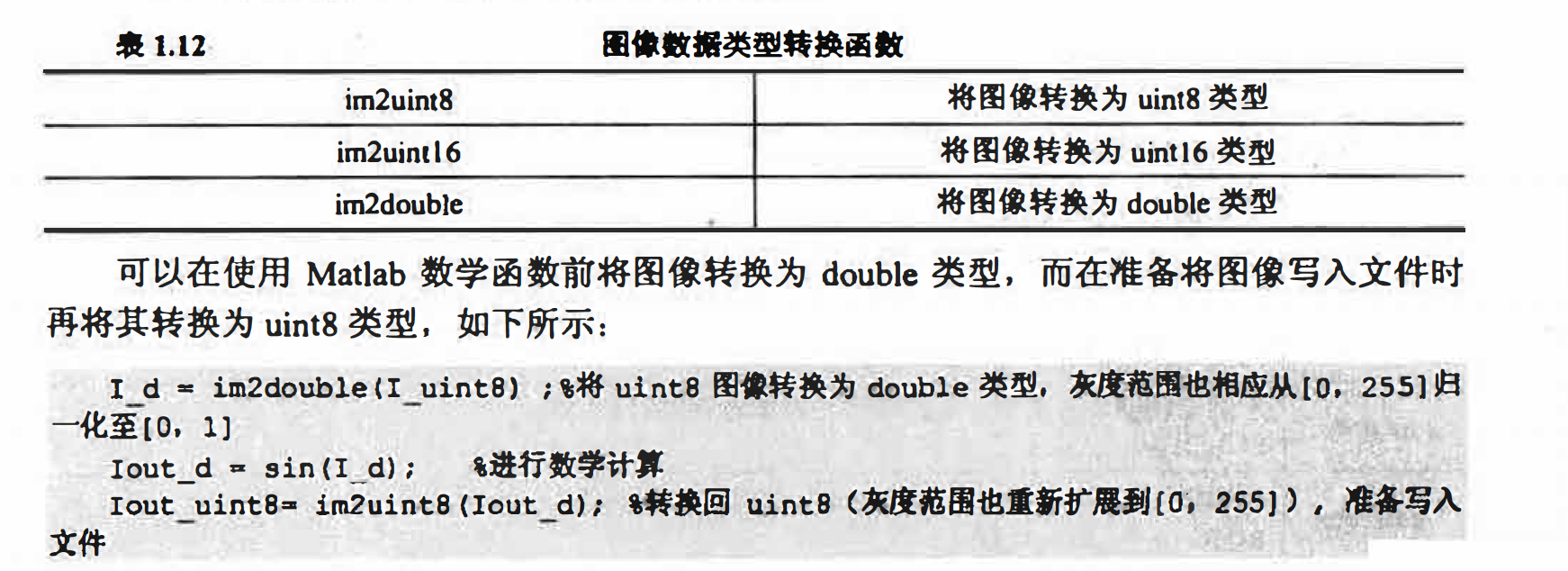图像的输入输出和显示

matlab可以处理以下的图像文件类型： BMP、HDF、JPEG、PCX、TIFF、XWD、ICO、
获得数字图像的相关信息．

型为：
A =imread(FileNAME, FMT);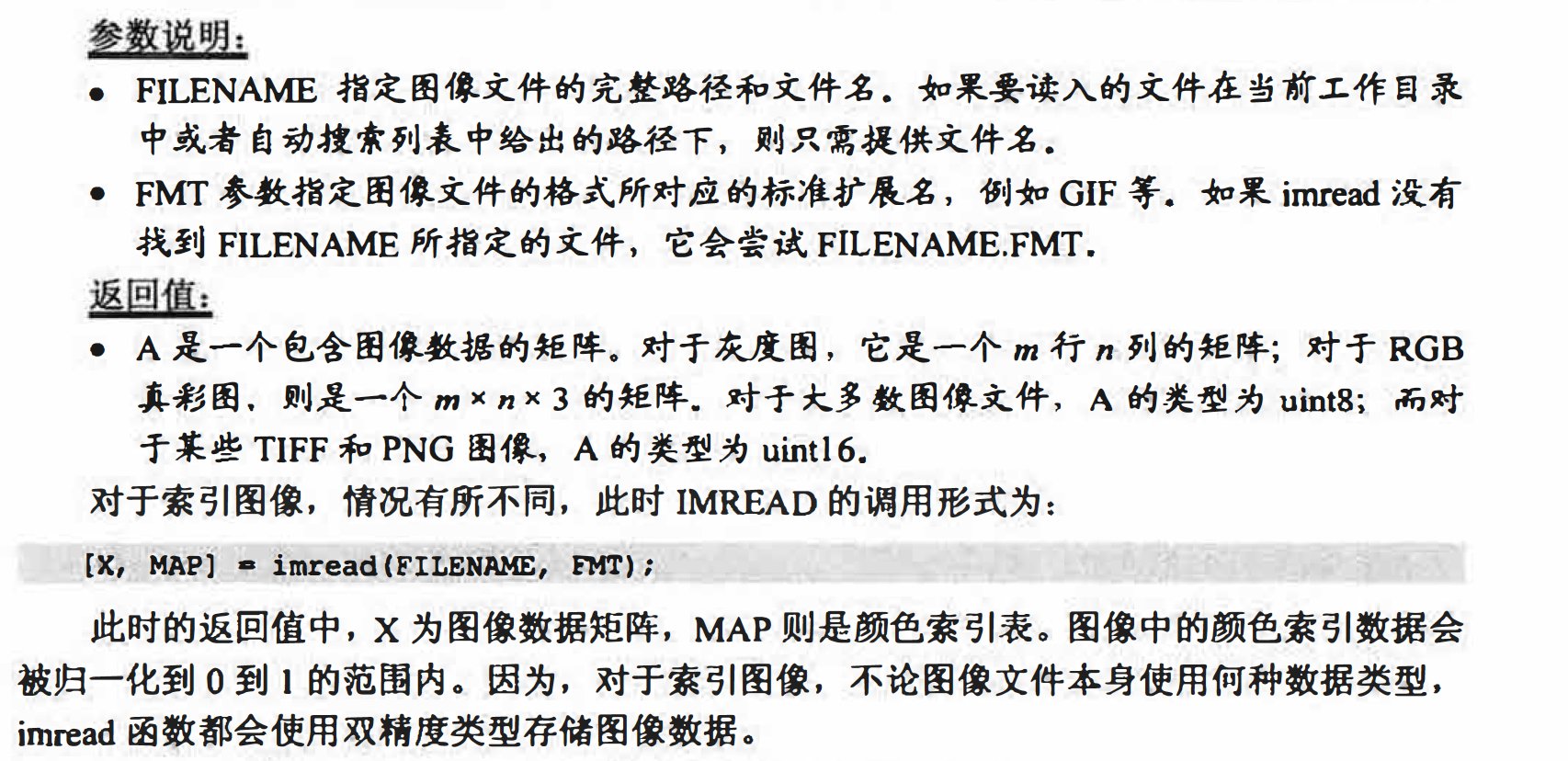2. IMWRITE函数
imwrite函数用于将指定的图像数据写入文件中，通过指定不同的保存文件扩展名，起到
图像格式转换的作用〈参见例2.4 ）. 其调用格式为：
imwite(A, FileName，FMT);
• FILENAME参数指定文件名（不必包含扩展名）．
• FMT参数指定保存文件所采用的格式．

存储索引图像时，还需要一并存储颜色索引表，则此时IMWRITE函数的使用方法应为：
imwrite(A, HAP, FILENAME, FMT);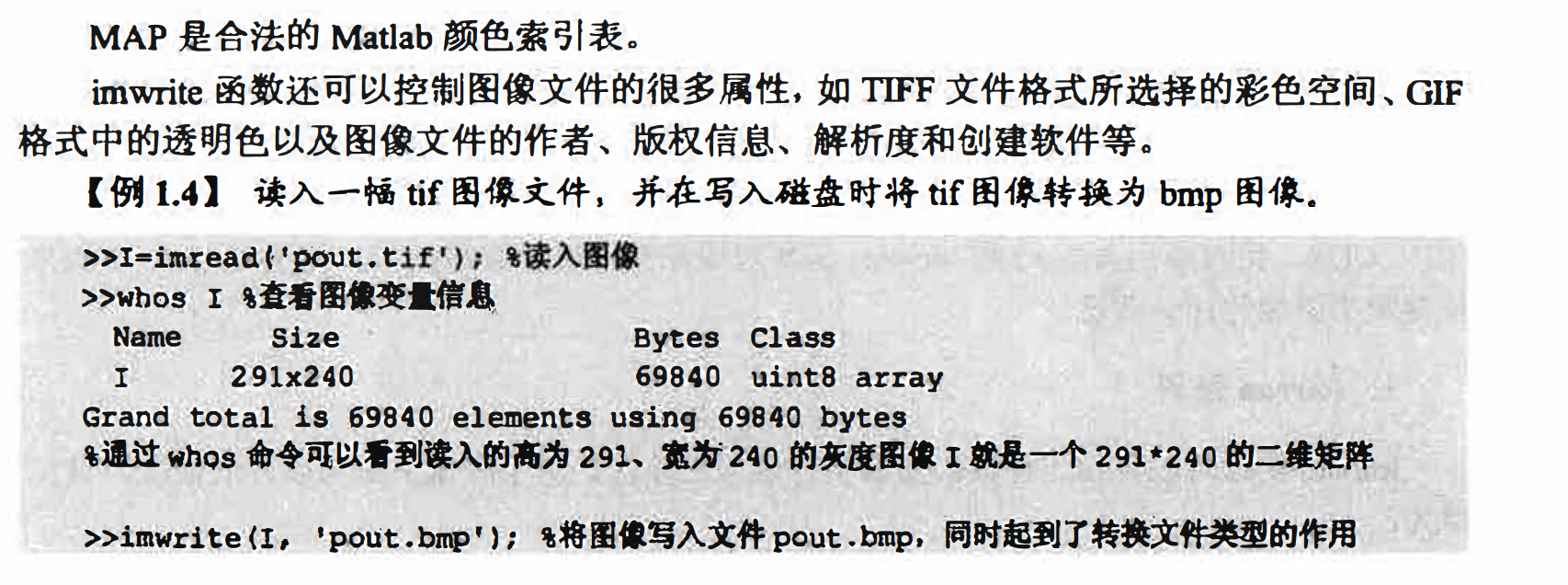3. imfinfo函数
imfinfo函数可以读取图像文件中的某些属性信息〈比如修改日期、大小、
格式、高度、宽度、色深、颜色空间、存储方式等。其调用格式为：
imfinfo(FileName,FMT);
参数说明
• FILENAME 参数指定文件名．
• FMT 参数是可选参数， 用于指定文件格式．

图像的显示
一般使用imshow函数来显示图像， 该函数可以创建一个图像对象， 并可以自动设置图
像的诸多属性，从而简化编程操作。这里介绍imshow函数的几种常见调用方式．

1.imshow函数
imshow函数用于显示工作区或图像文件中的图像．在显示的同时可控制部分效果〈参见
例12.6）， 常用的调用形式为：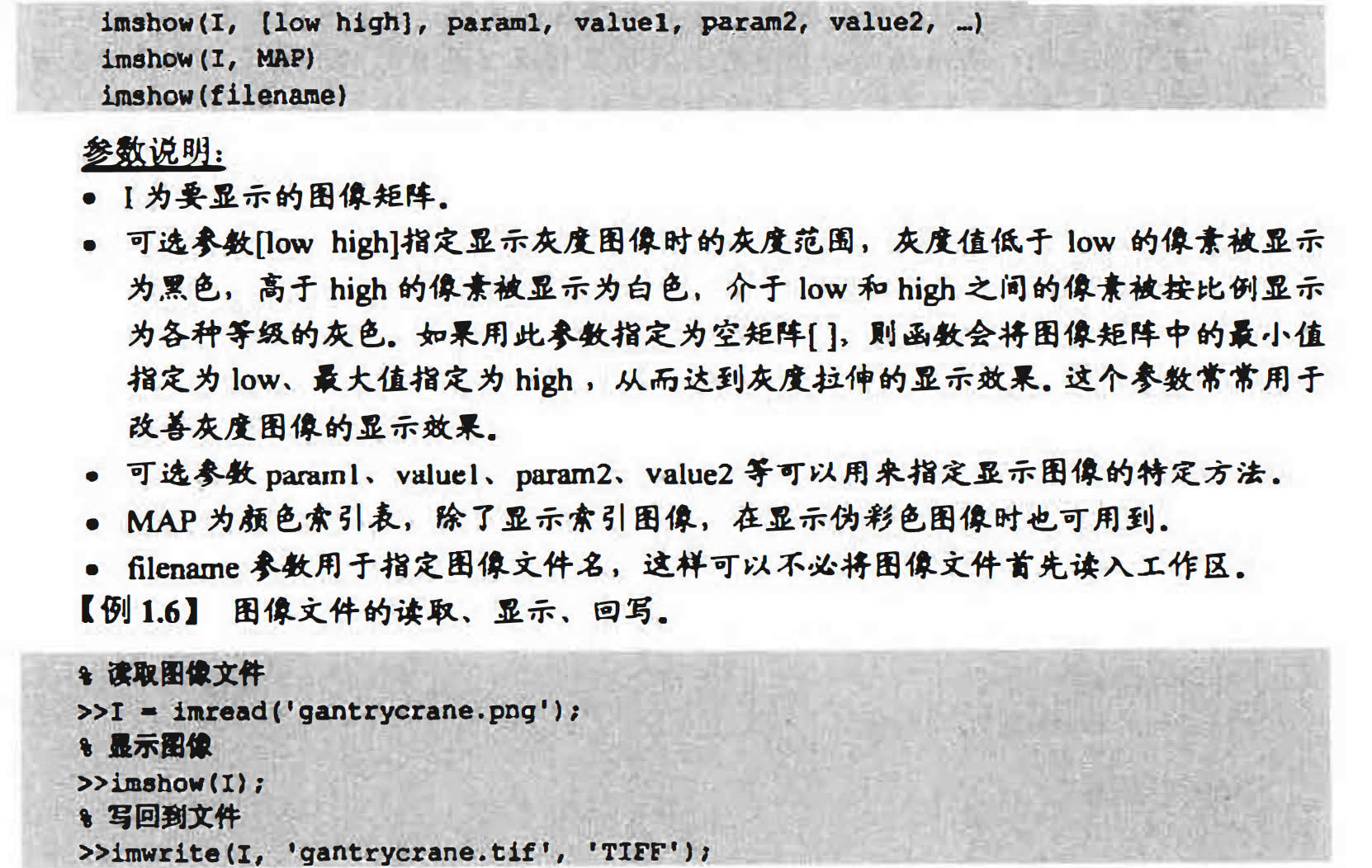2.多帧图像的显示
在显示多帧图像时，可以显示多帧中的一帧，或者将它们显示在同一个窗口内，也可以
将多帧图像转化成电影播放出来． 这3种方式的实现如例1.8所示。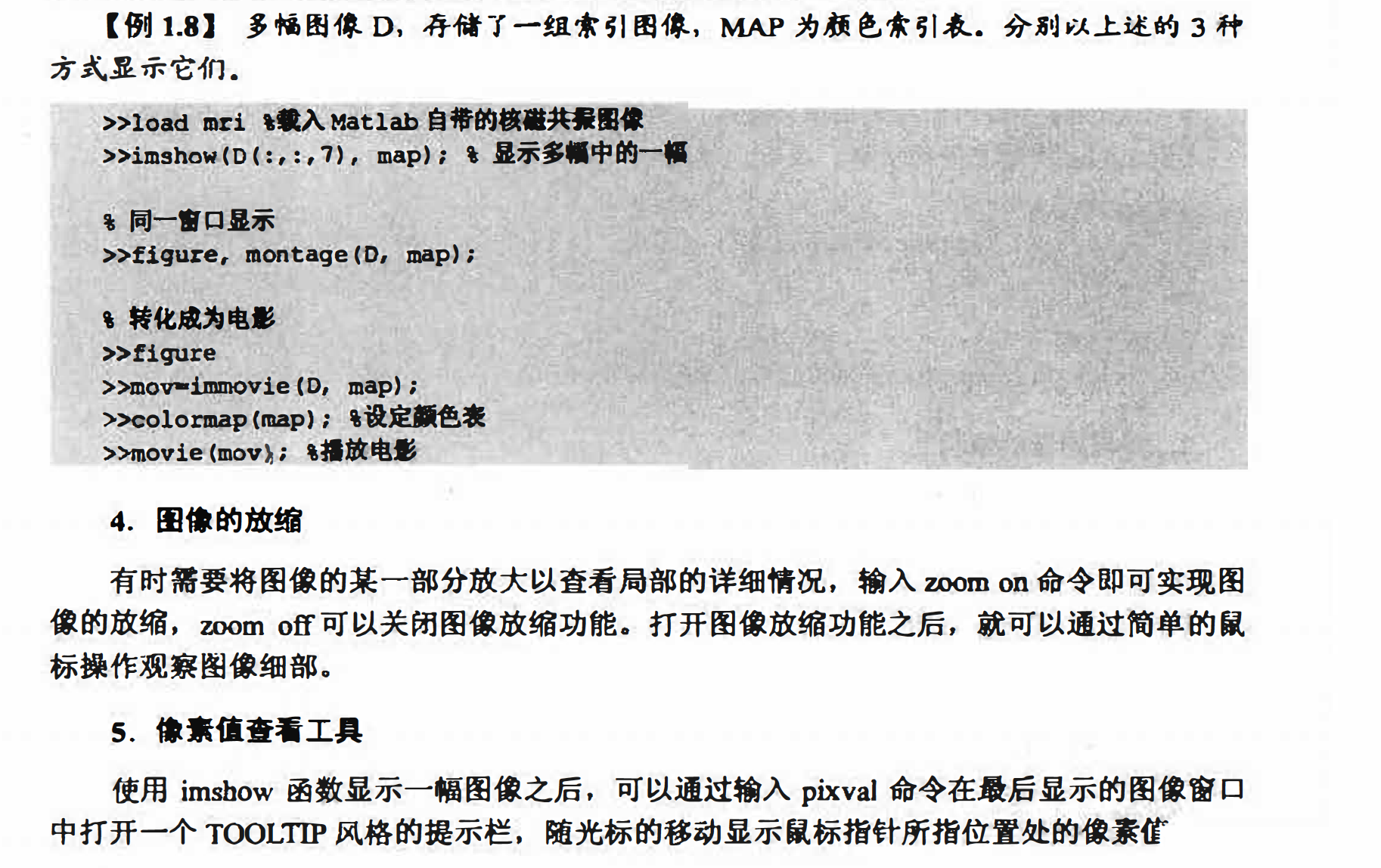展开全文图像处理 matlab 数据 class
• matlab代码，用于图像处理放向研究。把图像变成变灰度图像，索引图像或二值图像。
• 彩色图像：每个像素由R、G、B三个分量表示，每个通道取值范围0~255。数据类型一般为8位无符号整形。...索引图像：类似于查字典，为了解决彩色图像消耗空间大的问题，一般应用于色彩构成比较简单的场景...
彩色图像：每个像素由R、G、B三个分量表示，每个通道取值范围0~255。数据类型一般为8位无符号整形。

灰度图像：每个像素只有一个采样颜色的图像，这类图像通常显示为从最暗黑色到最亮的白色的灰度。

二值图像（黑白图像）：每个像素点只有两种可能，0和1.0代表黑色，1代表白色。数据类型通常为1个二进制位。

索引图像：类似于查字典，为了解决彩色图像消耗空间大的问题，一般应用于色彩构成比较简单的场景。

索引图像包括一个数据矩阵X，一个颜色映像矩阵Map。其中Map是一个包含三列、若干行的数据阵列，其中每个元素的值均为[0, 1]之间的双精度浮点型数据。Map矩阵的每一行分别表示红色、绿色和蓝色的颜色值。在MATLAB中，索引图像是从像素值到颜色映射表值的“直接映射”。像素颜色由数据矩阵X作为索引指向矩阵Map进行索引，例如，值1指向矩阵Map中的第一行，值2指向第二行，以此类推。

补充一张图帮助理解：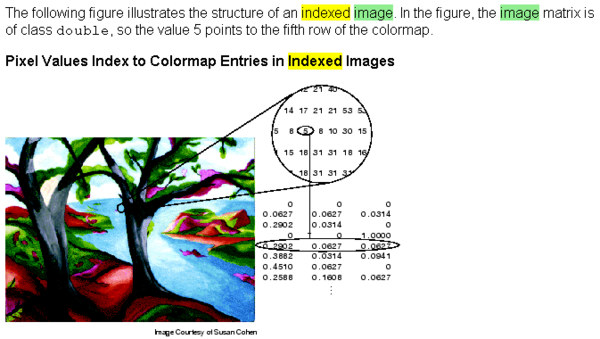图中圆圈内的就是索引图像的索引表，下面的就是其对应RGB颜色表。

图中小圆圈处的索引号是5，对应RGB颜色的第5行，所以该处RGB颜色实际是0.2902 0.0627 0.0627

索引图像的作用就是体积小，方便传输，只需要把索引表传输过去，接收方用对应的RGB颜色表还原就行。

[X,map] = rgb2ind(RGB,n)

使用最小方差量化和抖动将 RGB 图像转换为索引图像 X。map 最多包含 n 个颜色。n 必须小于或等于 65,536。
function IndexImg(Imgname)%索引图像
[I_index64,Map64] = rgb2ind(I_rgb,64);
[I_index256,Map256] = rgb2ind(I_rgb,256);

figure(1)
subplot(2,1,1),imagesc(I_rgb),title('Origin');
subplot(2,1,2),imagesc(I_index64),colormap(Map64),title('Index_Img64');

figure(2)
subplot(2,1,1),imagesc(I_rgb),title('Origin');
subplot(2,1,2),imagesc(I_index256),colormap(Map256),title('Index_Img256');

end

Map64对应64种颜色！

Map64 =

0.0863    0.0902    0.1020
0.5686    0.5255    0.3569
0.2078    0.2941    0.4510
0.7647    0.7176    0.5529
0.3137    0.2980    0.2039
0.3412    0.5216    0.7725
0.8667    0.8431    0.7529
0.2471    0.3922    0.6431
0.2157    0.2118    0.1490
0.4667    0.6078    0.8078
0.4314    0.4000    0.2667
0.6196    0.7137    0.8588
0.3686    0.3961    0.4510
0.1529    0.2078    0.2824
0.5176    0.5412    0.5843
0.4667    0.6235    0.8745
0.7020    0.7765    0.8824
0.2431    0.2980    0.3647
0.8706    0.9255    0.9843
0.4000    0.5647    0.8353
0.2784    0.4667    0.7412
0.2196    0.3333    0.5412
0.5020    0.4627    0.3020
0.1294    0.1490    0.1804
0.6196    0.5843    0.4275
0.6588    0.6353    0.5608
0.7490    0.8353    0.9843
0.4784    0.5608    0.7412
0.5412    0.6588    0.8784
0.3725    0.3373    0.2157
0.3843    0.4627    0.6275
0.6039    0.6510    0.7569
0.5686    0.7020    0.9412
0.1882    0.2431    0.3490
0.4588    0.4549    0.4471
0.2784    0.2549    0.1843
0.6824    0.7804    0.9765
0.2902    0.3412    0.4510
0.4157    0.4039    0.3490
0.3608    0.5569    0.9294
0.2824    0.3725    0.5490
0.1490    0.1490    0.1255
0.7529    0.7412    0.7020
0.3059    0.4157    0.6353
0.3255    0.3373    0.3529
0.3490    0.4667    0.7020
0.4039    0.5412    0.7647
0.1216    0.1686    0.2471
0.5529    0.5294    0.4431
0.8157    0.8745    0.9843
0.4431    0.4627    0.5412
0.7961    0.8510    0.9059
0.6941    0.6314    0.4392
0.2275    0.2471    0.2784
0.3059    0.3098    0.2745
0.5373    0.6667    0.9333
0.2000    0.2157    0.2157
0.5294    0.6392    0.8078
0.4941    0.4667    0.3686
0.3765    0.3490    0.2706
0.9529    0.9725    0.9882
0.4549    0.6196    0.9725
0.6235    0.7294    0.9490
0.3725    0.4196    0.5412


K>> size(Map64)

ans =

64     3


K>> size(I_index256)
ans =

    1080        1920


输出图片：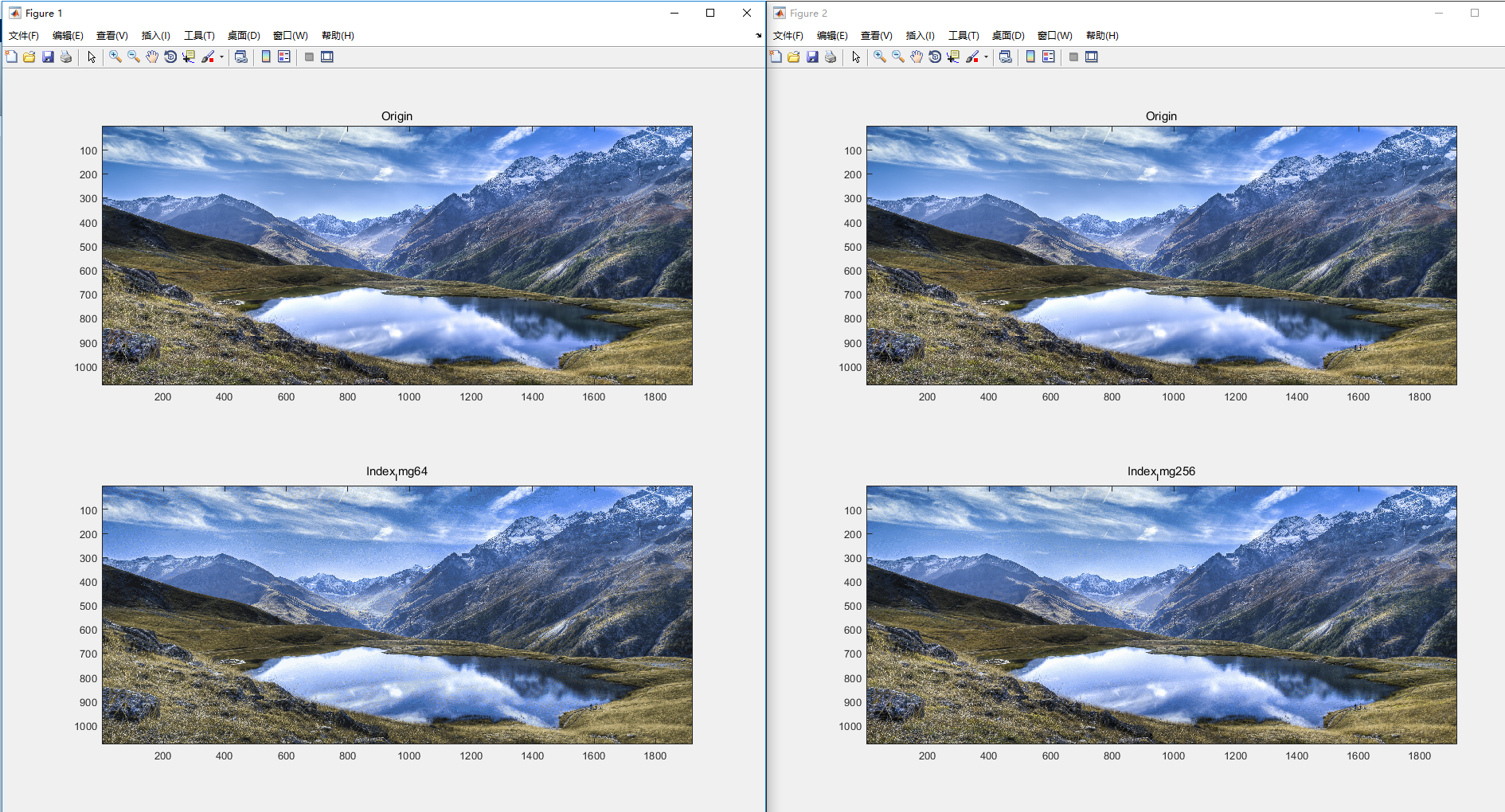处理图像的函数

其中用到的工具箱函数：

函数
目的
dither
采用抖动从RGB图像创建索引图像
grayslice
采用阈值处理从灰度图像创建索引图像
gray2ind
从灰度图像创建索引图像
ind2gray
从索引图像创建灰度图像
rgb2ind
从RGB图像创建索引图像
ind2rgb
从索引图像创建RGB图像
rgb2gray

从RGB图像创建灰度图像

im2bw
从灰度、索引、RGB图象创建二值图
mat2gray
从数据矩阵创建灰度图像

展开全文• 一、图像处理预备知识1、图像处理(image processing)用计算机对图像进行分析，以达到所需结果的技术。又称影像处理。图像处理一般指数字图像处理。在计算机中，按照颜色和灰度的多少可以将图像分为二值图像、灰度...
一、图像处理预备知识

1、图像处理(image processing)

用计算机对图像进行分析，以达到所需结果的技术。又称影像处理。图像处理一般指数字图像处理。

在计算机中，按照颜色和灰度的多少可以将图像分为二值图像、灰度图像、索引图像和真彩色RGB图像四种基本类型。

2、图像处理常用方法

1）图像变换

为了用正交函数或正交矩阵表示图像而对原图像所作的二维线性可逆变换。一般称原始图像为空间域图像，称变换后的图像为转换域图像，转换域图像可反变换为空间域图像。

它可以分为四个部分：空域变换等维度算法，空域变换变维度算法，值域变换等维度算法和值域变换变维度算法。其中空域变换主要指图像在几何上的变换，而值域变换主要指图像在像素值上的变换。等维度变换是在相同的维度空间中，而变维度变换是在不同的维度空间中，例如二维到三维，灰度空间到彩色空间。

常用的有三种变换方法：傅里叶变换、沃尔什-阿达玛变换、离散卡夫纳－勒维变换。这里只讲傅里叶变换。

2）图像增强和复原

图像增强不增加图片信息，只对感兴趣的特征进行强调突出，增加辨别能力。根据人的主观评定，具有一定的人为畸变。一般通过空间域处理和频率域处理两种方法。

图像复原技术的主要目的是以预先确定的目标来改善因散焦、像物相对运动、成像器材的图固有缺陷和外部干扰导致退化的图像。

图像增强和复原的目的是为了提高图像的质量，如去除噪声，提高图像的清晰度等。图像增强主要是一个主观过程，而图像复原则大部分是一个客观的过程。

3）图像压缩

图像压缩是一种减少描绘一幅图像所需数据量的技术和科学，它是数字图像处理领域中最有用和商业上最成功的技术之一。

图像压缩既可以是无损压缩，没有信息损失；也可以是有损压缩，不能通过解压恢复原图，牺牲准确度换来压缩能力的增加。

4）图像分割

图像分割是数字图像处理中的关键技术之一。分割将图像细分为构成它的子区域或物体。细分程度取决于要解决的问题。即将图像中有意义的特征部分提取出来，其有意义的特征有图像中的边缘、区域等，这是进一步进行图像识别、分析和理解的基础。

5）图像描述

图像描述是图像识别和理解的必要前提。作为最简单的二值图像可采用其几何特性描述物体的特性，一般图像的描述方法采用二维形状描述，它有边界描述和区域描述两类方法。

6）图像识别

图像经过某些预处理（增强、复原、压缩）后，进行图像分割和特征提取，从而进行判决分类。

二、MATLAB简介

Matlab是一个高级的矩阵/阵列语言，它包含控制语句、函数、数据结构、输入和输出和面向对象编程特点。用户可以在命令窗口中将输入语句与执行命令同步，也可以先编写好一个较大的复杂的应用程序（M文件）后再一起运行。

三、MATLAB图像处理代码

1、图像读取及保存

MATLAB的基本数据单位是矩阵，一幅图像可定义为一个二维函数f(x,y)，其中x和y是空间（平面）坐标，而在任何一对空间坐标(x,y)处的幅值f称为图像在该点处的强度或灰度。当x，y和灰度值f是有限的离散数值时，我们称该图像为数字图像。在MATLAB中，数字图像就是一个个矩阵。

1）图像读取

举例：

2）图像保存

函数：imwrite(OutImg, ‘图像文件’)：输出内存中图像数据到文件

举例：

imwrite(OutImg,'d:\photo\yn_ln.jpg'); %将OutImg矩阵表示的图像存储到电脑D盘photo文件夹中，并命名为yn_ln的.jpg格式的图像。

3）图像显示

函数：imshow(ImgData) ：将图像文件显示到屏幕
subplot(行数，列数，区域索引);

举例：

subplot(1,1,1);imshow(InImg); %将InImg图像文件显示到屏幕上指定位置

4）图像转换

I=rgb2gray(rgbImg)： 将彩色图像转换为灰度图像，rgbImg为彩色图像bw=im2bw(Img,level)： 将灰度图像转换为二值图像，Img为灰度图像，level为0~1的灰度级阈值；原图灰度图像                                                         二值图像

2、图像增强

1）数字图像灰度增强

J=imadjust(I,[low high], [bottom top],gamma): 对灰度图像进行直接灰度变换；I为增强前灰度图像，J为增强后的灰度图像，[low high]增强前灰度级范围，[bottom top]增强后灰度级范围

举例：

clear;
I=rgb2gray(InImg);
bw1=im2bw(I,0.8);
bw2=im2bw(J,0.8);
bw2=medfilt2(bw2);
subplot(1,2,1);imshow(bw1);
subplot(1,2,2);imshow(bw2);增强前                                                                                              增强后

2）数字图像空域滤波

S=medfilt2(J): 对图像进行中值滤波
举例：

M=0;V=0.01;
J1=imnoise(I, 'gaussian',M,V);
S=medfilt2(J1);
subplot(1, 2, 1); imshow(J1);
subplot(1, 2, 2); imshow(S);高斯噪声                                                                                        中值滤波

3）数字图像频域滤波

F=fft2(I): 2D 傅里叶正变换

Pha=angle(F): 计算相位谱

R= abs(F): 计算功率谱F1=real(F): 获得傅里叶变换实部分量F2=imag(F): 获得傅里叶变换虚部分量fftshift(F): 移动傅里叶频谱中心至零频率

I=ifft2(F): 2D 傅里叶逆变换

举例：（1）

I=BWBand(21,31);
F=fft2(I);                        %傅里叶正变换
F=fftshift(F);
L=abs(F);
L= mat2gray(L);
I3=imrotate(BWBand(21, 31),30);
F3=fft2(I3);
F3=fftshift(F3);
L3=abs(F3);
L3= mat2gray(L3);
subplot(141); imshow(I);
title('原图');
subplot(142); imshow(I3);
title('旋转30度图');
subplot(143); imshow(L);
title('功率谱图');
subplot(144); imshow(L3);

title('旋转30度功率谱图');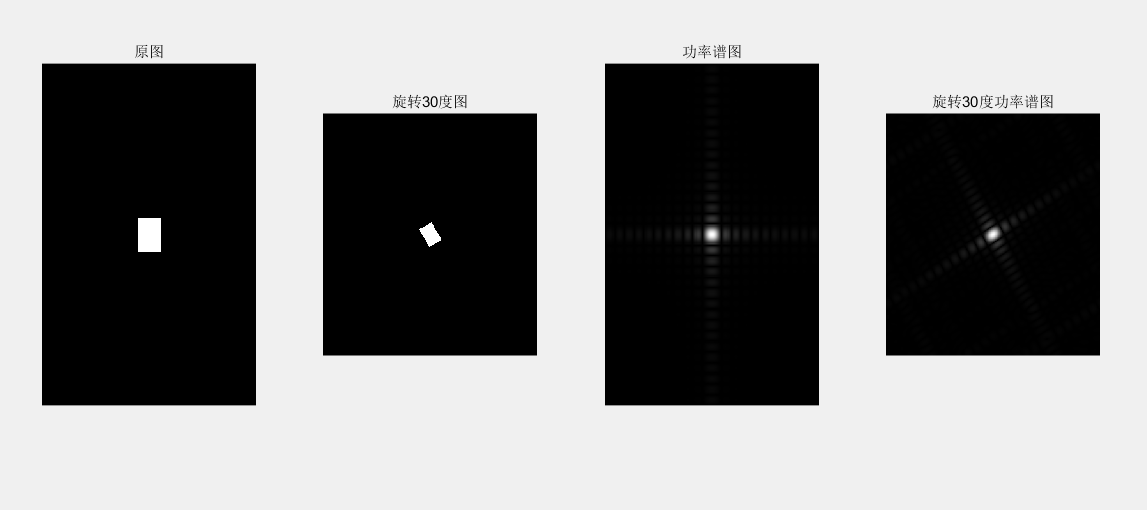subplot(2, 2, 1); imshow(InImg);
title('原彩色图');
I=rgb2gray(InImg);
J1=imnoise(I, 'salt & pepper',0.01);
subplot(2, 2, 2); imshow(J1);
title('椒盐噪声污染图');
[f1, f2]=freqspace([199 200],'meshgrid');        %产生等间隔采样的频率响应
R=sqrt(f1.^2+ f2.^2);                   %计算功率谱
Hd=ones([199 200]);
Hd(R>0.5)=0;                                   %产生理想的带通滤波器，频率带宽[0.1 0.5]
win= fspecial('gaussian',[199 200],2); %采用高斯函数生成同样大小的窗函数
win= win./max(win(:));                       %窗口函数归一化
H=fwind2(Hd,win);                           %利用窗口函数产生实际的带通滤波器
subplot(2, 2, 3); freqz2(H);
ff= imfilter (J1,H);
subplot(2, 2, 4); imshow(ff);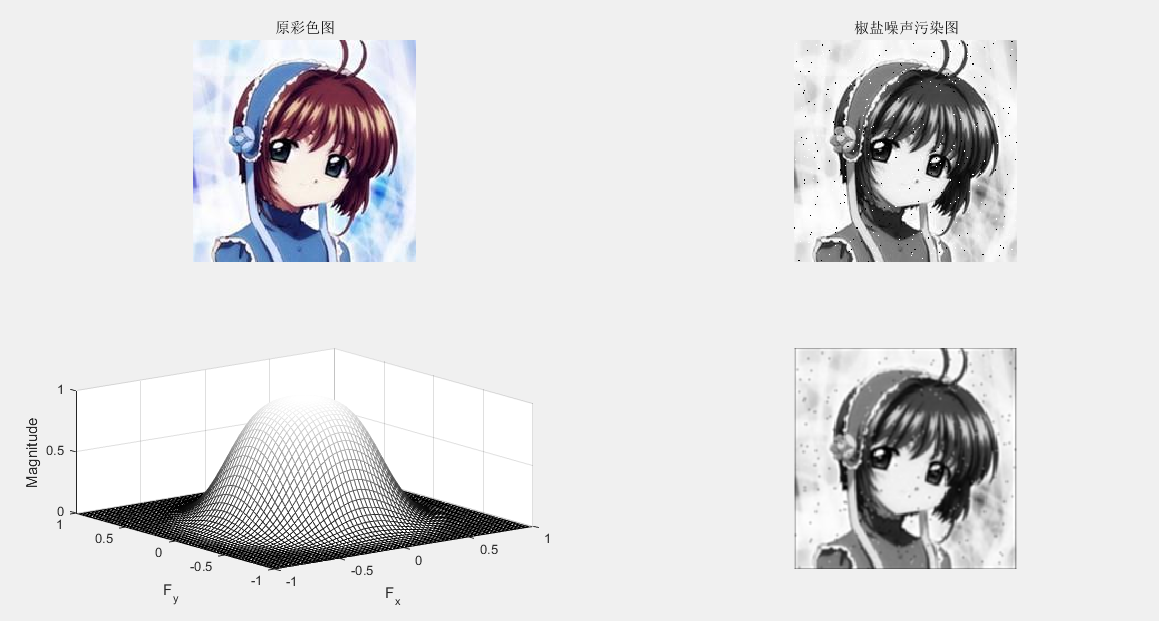3、图像复原

图像滤波：
J = WIENER2(I,[M N]): 对灰度图像实施维纳自适应滤波 ，[M N]像素领域大小C=conv2(A,B) : 计算矩阵 A 和 B 的卷积

举例：

I=rgb2gray(InImg);
J1=imnoise(I, 'gaussian');
B1=[0 0.2 0;0.2 0.2 0.2; 0 0.2 0];   %平滑滤波器
H = fspecial('average',[5 5]);
Blurred1 = imfilter (J1,H);
H = fspecial('gaussian',[5 5]);
Blurred2 = imfilter (J1,H);
K = wiener2(J1,[5 5]);
subplot(1, 5, 1); imshow(J1) ; %显示噪声图像
title('高斯噪声图像');
subplot(1, 5, 2); imshow(Blurred1) ; %显示均值滤波图像
title('均值滤波图像');
subplot(1, 5, 3); imshow(Blurred2) ; % 显示高斯滤波图像
title('高斯滤波图像');
subplot(1, 5, 4); imshow(K);% 显示维纳滤波器滤波图像
title('维纳滤波器滤波图像');
C1=conv2(J1,B1, 'same'); %对加噪后的图像平滑滤波
C1=mat2gray(C1); %将矩阵转换为灰度图像
subplot(1, 5, 5); imshow(C1);% 显示平滑图像
title('平滑图像');4、图像压缩

哈夫曼编码：无损压缩

步骤：1）缩减信源 2）尽可能少的调动信源符号排序 3）规定大概率事件编码

clear
close all;  %定义HufData/Len为全局变量的结构体
global HufData;
global Len;
disp('计算机正在准备输出霍夫曼编码结果,请耐心等待……'); %原始码字的灰度
a=rgb2gray(a);
figure;
subplot(1,2,1); imshow(a);
%取消坐标轴和边框
axis off
box off
title('Tokyo Towers','fontsize',13);
subplot(1,2,2);
axis off
box off
imhist(a);
title('图像灰度直方图','fontsize',13);%图像的灰度统计
GrayStatistics=imhist(a);
GrayStatistics=GrayStatistics';
GrayRatioo=GrayStatistics/sum(GrayStatistics);
GrayRatioNO=find(GrayRatioo~=0);
GrayRatioyes=find(GrayRatioo==0);
Len=length(GrayRatioNO);  %初始化灰度集，防止系统随即赋予其垃圾值
GrayRatio=ones(1,Len);
for i=1:Len
GrayRatio(i)=GrayRatioo(i);
end
GrayRatio=abs(sort(-GrayRatio));
for i=1:Len
HufData(i).value=GrayRatio(i); %将图像灰度概率赋予结构体
end  % 霍夫曼编码/霍夫曼编码
HuffmanCode(Len); %输出码字
lavg1=0;
for i=1:Len
tmpData=HufData(i).code
str='';
for j=1:length(tmpData) ;
str=strcat(str,num2str(tmpData(j)));
disp(strcat('a','= ',str));
end
len1=length(tmpData) ;
lavg1=lavg1+GrayRatio(1,i)*len1;

end
ratio_delet=8/lavg1;
str='';
str=strcat(str,num2str(ratio_delet));

disp(strcat('压缩率','= ',str))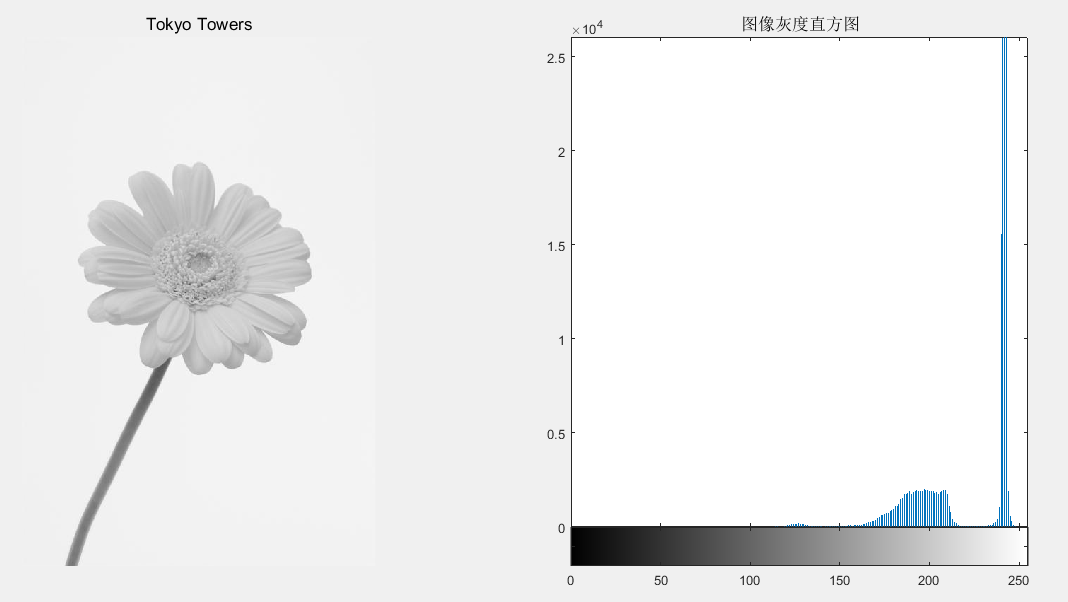5、图像分割（边缘提取）

1）图像几何纠正、边缘检测

B=imresize(A,m,type): 图像大小调整

B=imrotate(A,angle,method): 图像旋转调整

最近邻插值法：将变换后的图像中的原像素点最邻近像素的灰度值赋给原像素点的方法
双线性插值法：核心思想是在两个方向分别进行一次线性插值。

举例：

I=rgb2gray(InImg);
B1=imresize(I,1.5, 'nearest');
B2=imresize(I,1.5, 'bilinear');
C1= imrotate (I,45, 'nearest');
C2= imrotate (I,45, 'bilinear');
subplot(231);imshow(I) ;%显示原始图像于
title('原始图像');
subplot(232); imshow(B1) ;%显示调整图像(最近邻插值)
title('最近邻插值图像');
subplot(233); imshow(B2) ;% 显示调整图像(双线性插值)
title('双线性插值图像');
subplot(234); imshow(C1);% 显示旋转图像(最近邻插值)
title('旋转最近邻插值图像');
subplot(235); imshow(C2);% 显示旋转图像(双线性插值)
title('旋转双线性插值');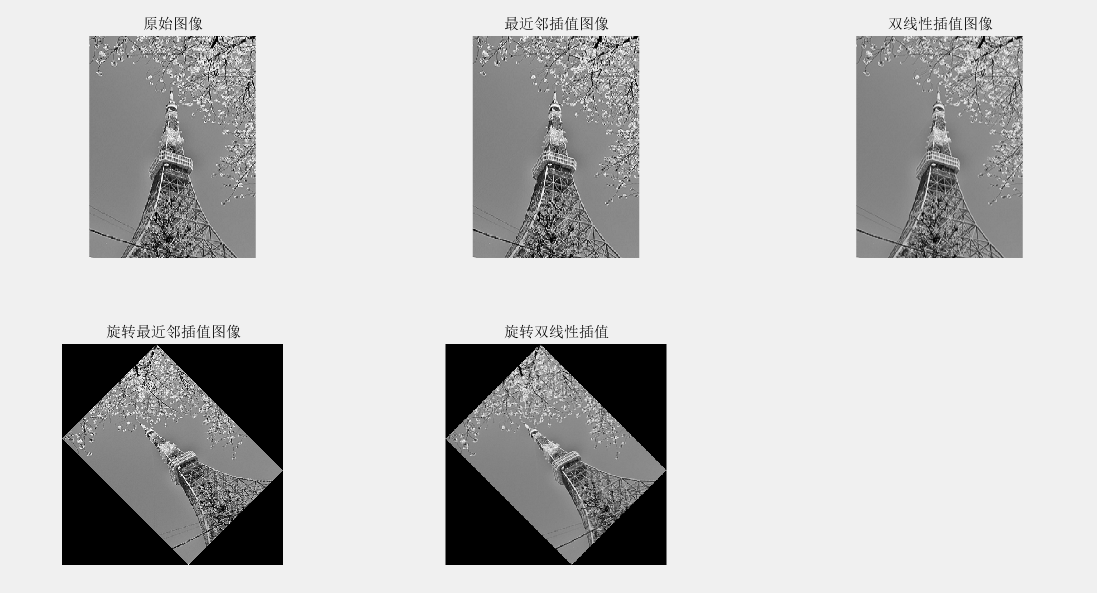2）边缘提取

BW=edge(I,method,thresh): 检测灰度图像的边缘

举例：

I=rgb2gray(InImg);
BW1= edge (I, 'sobel',0.1);%sobel边缘检测器
BW2= edge (I, 'prewitt',0.1);%prewitt边缘检测器
BW3= edge (I, 'roberts',0.1);%roberts边缘检测器
BW4= edge (I, 'log',0.01,2.0);%log边缘检测器
subplot(221); imshow(BW1) ;% 显示边缘图像
title('sobel边缘图像');
subplot(222); imshow(BW2) ;%显示边缘图像
title('prewitt边缘图像');
subplot(223); imshow(BW3) ;% 显示边缘图像
title('roberts边缘图像');
subplot(224); imshow(BW4) ;% 显示边缘图像
title('log边缘图像');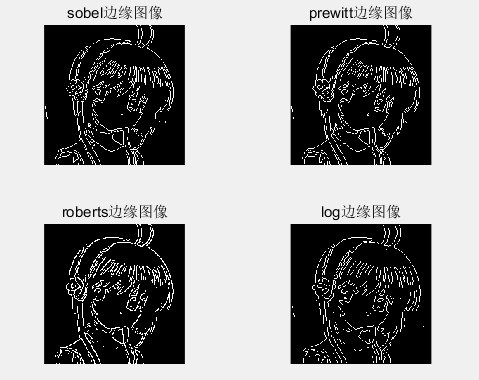边缘提取


展开全文• 本文阐述了一种基于MATLAB 的数字图像处理系统设计，其中包括图像处理领域的大部分算法， 运用MATLAB图像处理工具箱对算法进行了实现，论述了利用系统进行图像显示、图形表换及图像处理过程，系统支持索引图像、...MATLAB 数字图像处理
• 在进行数字图像处理时，需要将图像转换成灰度图。如truecolor转化为灰度图，可用函数rgb2gray即可实现，语法也比较简单。如下： grayImage=rgb2gray(srcImage); 加载完图片后可使用imfinfo()函数查看图像信息，...
本篇只讲应用不讲原理

在进行数字图像处理时，需要将图像转换成灰度图。如truecolor转化为灰度图，可用函数rgb2gray即可实现，语法也比较简单。如下：
grayImage=rgb2gray(srcImage);

加载完图片后可使用imfinfo()函数查看图像信息，通过colorType判断图像是什么类型，如为indexed即图像是索引图像。在Matlab函数库中可查找到indexed图像到gray图像的转换函数。如下：I=ind2gray(X,map);
[X,map]=imread('filename');

然后将[X,map]带入ind2gray函数即可实现索引图像向灰度图像的转换。

同样，对于索引图像向真彩图的转换可使用ind2rgb函数进行
RGB=ind2rgb(X,map)；
方法同上，不再赘述。grayImage=rgb2gray(srcImage);
展开全文索引图像
• 原文地址：Matlab图像处理函数汇总 作者：mimi 图像的变换  ① fft2：fft2函数用于数字图像的二维傅立叶变换，如：i=imread('104_8.tif'); j=fft2(i); ②ifft2:：ifft2函数用于数字图像的二维傅立叶反变换，如...

原文地址：Matlab图像处理函数汇总

作者：mimi

图像的变换
j=fft2(i);
②ifft2:：ifft2函数用于数字图像的二维傅立叶反变换，如：
j=fft2(i);
k=ifft2(j);

噪声生成函数
① imnoise：用于对图像生成模拟噪声，如：
j=imnoise(i,'gaussian',0,0.02);%模拟高斯噪声

定义滤波器
② fspecial：用于产生预定义滤波器，如：
h=fspecial('sobel');%sobel水平边缘增强滤波器
h=fspecial('gaussian');%高斯低通滤波器
h=fspecial('laplacian');%拉普拉斯滤波器
h=fspecial('log');%高斯拉普拉斯（LoG）滤波器
h=fspecial('average');%均值滤波器

图像的增强
①直方图：imhist函数用于数字图像的直方图显示，如：
imhist(i);
②直方图均化：histeq函数用于数字图像的直方图均化，如：
j=histeq(i);
④对数变换：log函数用于数字图像的对数变换，如：
j=double(i);
k=log(j);
h=[1,2,1;0,0,0;-1,-2,-1];
j=filter2(h,i);
⑥线性滤波：利用二维卷积conv2滤波, 如:
h=[1,1,1;1,1,1;1,1,1];
h=h/9;
j=conv2(i,h);
⑦中值滤波：medfilt2函数用于图像的中值滤波，如：
j=medfilt2(i);
⑧锐化
（1）利用Sobel算子锐化图像, 如:
h=[1,2,1;0,0,0;-1,-2,-1];%Sobel算子
j=filter2(h,i);
（2）利用拉氏算子锐化图像, 如:
j=double(i);
h=[0,1,0;1,-4,0;0,1,0];%拉氏算子
k=conv2(j,h,'same');
m=j-k;

图像边缘检测
①sobel算子 如：
j = edge(i,'sobel',thresh)
②prewitt算子 如：
j = edge(i,'prewitt',thresh)
③roberts算子  如：
j = edge(i,'roberts',thresh)
④log算子  如：
j = edge(i,'log',thresh)
⑤canny算子 如：
j = edge(i,'canny',thresh)
⑥Zero-Cross算子 如：
j = edge(i,'zerocross',thresh)

形态学图像处理
①膨胀：是在二值化图像中“加长”或“变粗”的操作，函数imdilate执行膨胀运算，如：
b=[0 1 0;1 1 1;0 1 0];
c=imdilate(a,b);
②腐蚀：函数imerode执行腐蚀，如：
b=strel('disk',1);
c=imerode(a,b);
③开运算：先腐蚀后膨胀称为开运算，用imopen来实现，如：
b=strel('square',2);
c=imopen(a,b);
④闭运算：先膨胀后腐蚀称为闭运算，用imclose来实现，如：
b=strel('square',2);
c=imclose(a,b);

===========================================================
Matlab 图像处理相关函数命令大全
一、通用函数：
colorbar  显示彩色条
语法：colorbar colorbar('vert') colorbar('horiz') colorbar(h) h=colorbar(...) colorbar(...,'peer',axes_handle)
getimage 从坐标轴取得图像数据
语法：A=getimage(h) [x,y,A]=getimage(h) [...,A,flag]=getimage(h) [...]=getimage

imshow 显示图像
语法：imshow(I,n) imshow(I,[low high]) imshow(BW) imshow(X,map) imshow(RGB) imshow(...,display_option) imshow(x,y,A,...) imshow filename h=imshow(...)

montage 在矩形框中同时显示多幅图像
语法：montage(I) montage(BW) montage(X,map) montage(RGB) h=montage(...)

immovie 创建多帧索引图的电影动画
语法：mov=immovie(X,map) mov=immovie(RGB)

subimage 在一副图中显示多个图像
语法：subimage(X,map) subimage(I) subimage(BW)   subimage(RGB) subimage(x,y,...) subimage(...)

truesize 调整图像显示尺寸
语法：truesize(fig,[mrows mcols]) truesize(fig)

warp 将图像显示到纹理映射表面
语法：warp(X,map) warp(I ,n) warp(z,...) warp(x,y,z,...)   h=warp(...)

zoom 缩放图像
语法：zoom on zoom off zoom out zoom reset zoom zoom xon zoom yon zoom(factor) zoom(fig,option)

二、图像文件I/O函数命令
imfinfo  返回图形图像文件信息
语法：info=imfinfo(filename,fmt) info=imfinfo(filename)

imwrite  把图像写入（保存）图像文件中
语法：imwrite(A,filename,fmt) imwrite(X,map,filename,fmt) imwrite(...,filename) imwite(...,Param1,Val1,Param2,Val2...)

imcrop  剪切图像
语法：I2=imcrop(I) X2=imcrop(X,map) RGB2=imcrop(RGB) I2=imcrop(I,rect) X2=imcrop(RGB,rect) [...]=imcrop(x,y,...) [A,rect]=imcrop(...) [x,y,A,rect]=imcrop(...)

imresize  改变图像大小
语法：B=imresize(A,m,method)

imrotate  旋转图像
语法：B=imrotate(A,angle,method) B=imrotate(A,angle,method,'crop')

三、像素和统计处理函数
corr2  计算两个矩形的二维相关系数
语法：r=corr2(A,B)

imcontour 创建图像数据的轮廓图
语法：imcontour(I,n) imcontour(I,v) imcontour(x,y,...) imcontour(...,LineSpec) [C,h] =imcontour(...)

imfeature  计算图像区域的特征尺寸
语法：stats=imfeature(L,measurements) stats=imfeature(L,measurements,n)

imbist  显示图像数据的柱状图

impixel 确定像素颜色值
语法：P=impixel(I) P=impixel(X,map) P=impixel(RGB) P=impixel(I,c,r) P=impixel(X,map,c,r) P=impixel(RGB,c,r) [c,r,P]=impixel(...) P=impixel(x,y,I,xi,yi) P=impixel(x,y,RGB,xi,yi) P=impixel(x,y,X,map,xi,yi)
[xi,yi,P]=impixel(x,y,...)

improfile 沿线段计算剖面图的像素值
语法：c=improfile c=improfile(n) c=improfile(I,xi,yi) c=improfile(I,xi,yi,n)   [cx,cy,c]=improfile(...) [cx,cy,c,xi,yi]=improfile(...) [...]=improfile(x,y,I,xi,yi)   [...]=improfile(x,y,I,xi,yi,n)
[...]=improfile(...,method)

mean2 计算矩阵元素的平均值
语法：B=mean2(A)

pixval  显示图像像素信息
语法：pixval on

std2 计算矩阵元素的标准偏移
语法：b=std2(A)

四、图像分析函数：
edge 图像边缘检测
语法：BW=edge(I,'sobel') BW=edge(I,'sobel',thresh) BW=edge(I,'sobel',thresh,direction) [BW,thresh]=edge(I,'sobel',...) BW=edge(I,'prewitt') BW=edge(I,'prewitt',thresh) BW=edge(I,'prewitt',thresh,direction)
[BW,thresh]=edge(I,'prewitt',...)   BW=edge(I,'roberts') BW=edge(I,'roberts',thresh) [BW,thresh]=edge(I,'roberts',...) BW=edge(I,'log') BW=edge(I,'log',thresh) BW=edge(I,'log',thresh,sigma) [BW,threshold]=edge(I,'log',...)
BW=edge(I,'zerocross',thresh,h) [BW,thresh]=edge(I,'zerocross',...)
BW=edge(I,'canny') BW=edge(I,'canny',thresh) BW=edge(I,'canny',thresh,sigma) [BW,threshold]=edge(I,'canny',...)
qtgetblk  获取四叉树分解的块值
语法：[vals,r,c]=qtgetblk(I,S,dim) [vals,idx]=qtgetblk(I,S,dim)
qtsetblk 设置四叉树分解中的块值
语法：J=qtsetblk(I,S,dim,vals)

五、图像增强函数
histeq 用柱状图均等化增强对比
语法：J=histeq(I,hgram) J=histeq(I,n) [J,T]=histeq(I,...) newmap=histeq(X,map,hgram) newmap=histeq(X,map)
imnoise 增强图像的渲染效果
语法：J=imnoise(I,type) J=imnoise(I,type,parameters)
medfilt2 进行二维中值过滤
语法：B=medfilt2(A,[m n]) B=medfilt2(A) B=medfilt2(A,'indexed',...)
ordfilt2 进行二维统计顺序过滤
wiener2 进行二维适应性去噪过滤处理
语法：J=wiener2(I,[m  n],noise) [J,noise]=wiener2(I,[m n])

六、线性滤波函数
conv2 进行二维卷积操作
语法：C=conv2(A,B) C=conv2(hcol,hrow,A) C=conv2(...,'shape')
convmtx2 计算二维卷积矩阵
语法：T=convmtx2(H,m,n) T=convmtx2(H,[m n])
convn 计算n维卷积
语法：C=convn(A,B) C=convn(A,B,'shape')
filter2 进行二维线性过滤操作
语法：Y=filter2(h,X) Y=filter2(h,X,shape)
fspecial 创建预定义过滤器
语法：h=fspecial(type) h=fspecial(type,parameters)

七、线性二维滤波设计函数
freqspace 确定二维频率响应的频率空间
语法：[f1,f2]=freqspace(n) [f1,f2]=freqspace([m n]) [x1 ,y1]=freqspace(...,'meshgrid') f=freqspace(N) f=freqspace(N,'whole')
freqz2 计算二维频率响应
语法：[H,f1,f2]=freqz2(h,n1,n2) [H,fi,f2]]=freqz2(h,[n2,n1]) [H,fi,f2]]=freqz2(h,f1,f2]) [H,fi,f2]]=freqz2(h) [...]=freqz2(h,...,[dx dy]) [...]=freqz2(h,...,dx) freqz2(...)
fsamp2 用频率采样法设计二维FIR过滤器
语法：h=fsamp2(Hd) h=fsamp2(f1,f2,Hd,[m n])
ftrans2 通过频率转换设计二维FIR过滤器
语法：h=ftrans2(b,t) h=ftrans2(b)
fwind1 用一维窗口方法设计二维FIR过滤器
语法：h=fwind1(Hd,win) h=fwind1(Hd,win1,win2) h=fwind1(f1,f2,Hd,...)
fwind2 用二维窗口方法设计二维FIR过滤器
语法：h=fwind2(Hd,win) h=fwind2(f1,f2,Hd,win)

八、图像变换函数
dct2 进行二维离散余弦变换(反余弦变换用idct2)
语法：B=dct2(A) B=dct2(A,m.n) B=dct2(A,[m n])
dctmtx 计算离散余弦傅立叶变换
语法：D=dctmtx(n)
fft2 进行二维快速傅立叶变换(反变换用ifft2)
语法：Y=fft2(X) Y=fft2(X,m,n)
fftn 进行n维快速傅立叶变换(反变换用ifftn)
语法：Y=ffn(X) Y=fftn(X,siz)
fftshift 快速傅立叶变换的DC组件移到光谱中心
语法：Y=fftshift(X) Y=fftshift(X,dim)
phantom 产生一个头部幻影图像
语法：P=phantom(def,n) P=phantom(E,n) [P,E]=phantom(...)

九、边沿和块处理函数
bestblk 确定进行块操作的块大小
语法：siz=bestblk([m n],k) [mb,nb]=bestblk([m n],k)
blkproc 实现图像的显示块操作
语法：B=blkproc(A,[m n]),fun) B=blkproc(A,[m n],fun,P1,P2,...) B=blkproc(A,[m n],[mborder nborder],fun,...)
col2im 将矩阵的列重新组织到块中
语法：A=col2im(B,[m n],[mm nn],block_type) A=col2im(B,[m n],[mm nn])
colfilt 利用列相关函数进行边沿操作
语法：B=colfilt(A,[m n],block_type,fun) B=colfilt(A,[m n],block_type,fun,P1,P2,...) B=colfilt(A,[m n],[mblock nblock],...) B=colfilt(A,'indexed',...)
im2col 重调图像块为列
语法：B=im2col(A,[m n],block_type) B=im2col(A,[m n]) B=im2col(A,'indexed',...)
nlfilter 进行边沿操作
语法：B=nlfilter(A,[m n],fun) B=nlfilter(A,[m n],fun,P1,P2,...) B=nlfilter(A,'indexed',...)

十、二进制图像操作函数
applylut 在二进制图像中利用lookup表进行行边沿操作
语法：A=applylut(BW,LUT)
bwarea 计算二进制图像对象的面积
语法：total=bwarea(BW)
bweuler 计算二进制图像的欧拉数
语法：eul=bweuler(BW)
bwfill 填充二进制图像的背景色
语法：BW2=bwfill(BW1,c,r,n) BW2=bwfill(BW1,n) [BW2,idx]=bwfill(...) BW2=bwfill(x,y,BW1,xi,yi,n) [x,y,BW2,idx,xi,yi]=bwfill(...)   [BW2,idx]=bwfill(BW1,'holes',n)
bwlabel 标注二进制图像中已连接的部分
语法：L=bwlabel(BW,n) [L,num]=bwlabel(BW,n)
bwmorph 提取二进制图像的轮廓
语法：BW2=bwmorph(BW1,operation) BW2=bwmorph(BW1,operation,n)
bwperim 计算二进制图像中对象的周长
语法：BW2=bwperim(BW1) BW2=bwperim(BW1,CONN)
bwselect 在二进制图像中选择对象
语法：BW2=bwselect(BW1,c,r,n) BW2=bwselect(BW1,n) [BW2,idx]=bwselect(...) BW2=bwselect(x,y,BW1,xi,yi,n) [x,y,BW2,idx,xi,yi]=bwselect(...)
dilate 放大二进制图像
语法：BW2=dilate(BW1,SE) BW2=dilate(BW1,SE,alg) BW2=dilate(BW1,SE,...,n)
erode 弱化二进制图像的边界
语法：BW2=erode(BW1,SE) BW2=erode(BW1,SE,alg) BW2=erode(BW1,SE,...,n)
makelut 创建一个用于applylut函数的lookup表
语法：lut=makelut(fun,n) lut=makelut(fun,n,P1,P2,...)

十一、区域处理函数
roicolor 选择感兴趣的颜色区
语法:BW=roicolor(A,low,high) BW=rocicolor(A,v)
roifill 在图像的任意区域中进行平滑插补
语法:J=roifill(I,c,r) J=roifill(I) J=roifill(I,BW) [J,BW]=roifill(...) J=roifill(x,y,I,xi,yi) [x,y,J,BW,xi,yi]=roifill(...)
roifilt2 过滤敏感区域
语法:J=roifilt2(h,I,BW) J=roifilt2(I,BW,fun) J=roifilt2(I,BW,fun,P1,P2,...)
roipoly 选择一个敏感的多边形区域
语法:BW=roipoly(I,c,r) BW=roipoly(I) BW=roipoly(x,y,I,xi,yi) [BW,xi,yi]=roipoly(...) [x,y,BW,xi,yi]=roipoly(...)

十二、颜色映像处理函数
brighten 增加或降低颜色映像表的亮度
语法：brighten(beta) brighten(h,beta) newmap=brighten(beta) newmap=brighten(cmap,beta)
cmpermute 调整颜色映像表中的颜色
语法：[Y,newmap]=cmpermute(X,map) [Y,newmap]=cmpermute(X,map,index)
cmunigue 查找颜色映像表中特定的颜色及相应的图像
语法：[Y,newmap]=cmunigue(X,map) [Y,newmap]=cmunigue(RGB) [Y,newmap]=cmunique(I)
imapprox 对索引图像进行近似处理
语法：[Y,newmap]=imapprox(X,map,n)   [Y,newmap]=imapprox(X,map,tol) Y=imapprox(X,map,newmap) [...]=imapprox(...,dither_option)
rgbplot 划分颜色映像表
语法：rgbplot(cmap)

十三、颜色空间转换函数
hsv2rgb 转换HSV值为RGB颜色空间：M=hsv2rgb(H)
ntsc2rgb 转换NTSC值为RGB颜色空间：rgbmap=ntsc2rgb(yiqmap) RGB=ntsc2rgb(YIQ)
rgb2hsv 转换RGB值为HSV颜色空间：cmap=rgb2hsv(M)
rgb2ntsc 转换RGB值为NTSC颜色空间：yiqmap=rgb2ntsc(rgbmap) YIQ=rgb2ntsc(RGB)
rgb2ycbcr 转换RGB值为YCbCr颜色空间：ycbcrmap=rgb2ycbcr(rgbmap) YCBCR=rgb2ycbcr(RGB)
ycbcr2rgb 转化YCbCr值为RGB颜色空间：rgbmap=ycbcr2rgb(ycbcrmap) RGB=ycbcr2rgb(YCBCR)

十四、图像类型和类型转换函数
dither 通过抖动增加外观颜色分辨率转换图像
语法：X=dither(RGB,map) BW=dither(I)
gray2ind 转换灰度图像为索引图像
语法：[X,map]=gray2ind(I,n) [X,map]=gray2ind(BW,n)
grayslice 从灰度图像为索引图像
语法：X=grayslice(I,n) X=grayslice(I,v)
im2bw 转换图像为二进制图像
语法：BW=im2bw(I,level) BW=im2bw(X,map,level) BW=im2bw(RGB,level)
im2double 转换图像矩阵为双精度型
语法：I2=im2double(I1) RGB2=im2double(RGB1) I=im2double(BW) X2=im2double(X1,'indexed')
double 转换数据为双精度型
语法：double(X)
unit8 、unit16转换数据为8位、16位无符号整型： i=unit8(x) i=unit16(x)
im2unit8 转换图像阵列为8位无符号整型
语法：I2=im2unit8(I1) RGB2=im2unit8(RGB1) I=im2unit8(BW) X2=im2unit8(X1,'indexed')
im2unit16 转换图像阵列为16位无符号整型
语法：I2=im2unit16(I1) RGB2=im2unit16(RGB1) I=im2unit16(BW) X2=im2unit16(X1,'indexed')
ind2gray 把检索图像转化为灰度图像
语法：I=ind2gray(X,map)
ind2rgb  转化索引图像为RGB真彩图像
语法：RGB=ind2rgb(X,map)
isbw 判断是否为二进制图像
语法：flag=isbw(A)
isgray 判断是否为灰度图像
语法：flag=isgray(A)
isind 判断是否为索引图像
语法：flag=isind(A)
isrgb 判断是否为RGB真彩色图像
语法：flag=isrgb(A)
mat2gray 转换矩阵为灰度图像
语法：I=mat2gray(A,[amin amax]) I=mat2gray(A)
rgb2gray 转换RGB图像或颜色映像表为灰度图像
语法：I=rgb2gray(RGB) newmap=rgb2gray(map)
rgb2ind 转换RGB图像为索引图像
语法：[X,map]=rgb2ind(RGB,tol) [X,map]=rgb2ind(RGB,n) X=rgb2ind(RGB,map) [...]=rgb2ind(...,dither_option)

十五、新增图像处理工具箱函数
applycform 用于颜色空间变换 out=applyform(I,C)
bwboundaries 描绘二进制图像边界
语法： B=bwboundaries(BW) B=bwboundaries(BW,CONN) B=bwboundaries(BW,CONN,options) [BW,CONN,options] [BL]=bwboundaries(...) [BLNA]=bwboundaries()
bwtraceboundary 描述二进制图像中的物体
B=bwtraceboundary(BW,P,fstep) B=bwtraceboundary(BW,P,fstep,CONN) B=bwtraceboundary(...N,dir)
decorrstrech 对多通道图像进行去相关处理
语法：S=decorrstretch(I) S=decorrstretch(I,TOL)
dicomdict 获取或读取DICOM文件
语法：dicomdict('set',dictionary) dictionary=dicomdict('get')
getline 用鼠标选择ployline
语法：[x,y]=getline(fig) [x,y]=getline(ax) [x,y]=getline [x,y]=getline(...,'closed')
getpts 用鼠标选择像素点
语法：[x,y]=getpts(fig) [x,y]=getpts(ax) [x,y]=getpts
getrect 用鼠标选择矩阵
语法：rect=getrect(fig) rect=getrect(ax) rect=getrect(fig)
im2java2d 将图像转换为Java缓冲图像
语法：jimage=im2java2d(I) jimage=im2java2d(X,MAP)
imview 在图像与蓝旗中显示图像
语法：imview(I)   imview(RGB) imview(X,map) imview(I,range) imview(filename) imview(....'InitialMagnification',initial_mag) h=imview(...)    imview close all
ippl 检查IPPL的存在
语法：TF=ippl [TF B]=ippl
iptdemos 显示图像处理工具箱中的索引图像
lab2double、lab2unit16、lab2unit8 将L*a*b数据分别转换为双精度、16位数据、8位数据
makecform 创造一个色彩转换结构
unitlut 查找表中A像素值
语法：B=unitlut(A,LUT)
xyz2double、xyz2unit16 将颜色数据从XYZ转换到双精度、16进制。
语法：xyzd=xyz2double(XYZ) xyz16=xyz2unit16(xyz)


展开全文图像处理 matlab 编程 数据 函数
• 第一部分：MATLAB图像处理基础 第一章：MATLAB基础知识 第二章：MATLAB矩阵及其运算 第三章：MATLAB图像处理基础 第一章：MATLAB基础知识 1.1 MATLAB概述与桌面操作 1、matlab将数值分析、矩阵计算、科学...
• 下面来介绍一下如何在MATLAB中进行图像的索引和抖动转换，这是对于图像的例化输出很经典的操作，具体如下： 1、打开MATLAB软件，在其主界面的编辑器中写入下列代码： ...%显示索引图像如figure1所示。 imshow(...
• MATLAB中，基本数据结构为数列，大部分...这样就把图像变成了数列了，MATLAB就可以使用数学函数对图像进行处理了。 1.MATLAB图像表达方式 在MATLAB中，图像可以以两种方式表达，分别为像素索引和空间位置。 （1）
• 常见的5种图像表示方法:分别是二进制图像,索引图像,灰度图像,RGB图像和多帧图像 1.2.1二进制图像(二值图像) 通常由一个二维数组表示,一位表示一个像素.非0即1,0表示黑色,1表示白色 1.2.2 灰度图像(单色图像) ...matlab 数字图像处理
• matlab命令索引图像处理函数详解-Matlab图像处理工具箱函数详解汇总.doc 也上传一点资料供大家参考，希望对大家有帮助matlab
• 显示索引图像和灰度图像&gt;&gt; [X,map]=imread('trees.tif');&gt;&gt; gmap=rgb2gray(map);&gt;&gt; figure,imshow(X,map);&gt;&gt; figure,imshow(X,gmap);利用膨胀函数平移图像...matlab函数 数字图像处理函数
• 8.2.2 数字图像处理的主要内容 8.3 图像文件格式 8.4 图像类型 8.4.1 索引图像 8.4.2 灰度图像 8.4.3 RGB 图像 8.4.4 二值图像 8.4.5 图像序列 8.4.6 图形类型判断 8.5 图像类型转换 8.5.1 dither 函数 ...
• Figure,rgbcube(0,0,10); %从正面观察彩色立方体 figure,rgbcube(10,0,10); %从侧面观察彩色立方体 figure,rgbcube(10,10,10);...%rgb图像转换成8色索引图像，不采用抖动方式 [X1,map1]=rgb2ind(f,8,'n...
• 自己在学习过程中查阅资料整理了MATLAB图像处理工具箱中函数的名称列表，以及MathWorks中讲解各个函数使用的链接： 1.绘图工具箱 plot 基本绘图函数 mesh 绘制三维网格曲面 fplot 绘制数学函数图 ....matlab 图像处理
• 灰度图像灰度图像转化rgb2gray灰度图像转化rgb2ind灰度图像向索引图像的转换gray2ind将灰度图像转换为索引图像grayslice索引图像转换为灰度图像ind2gray索引图像转换为真彩色图像ind2rgb灰度图像转换为二值图像im2bw...
...